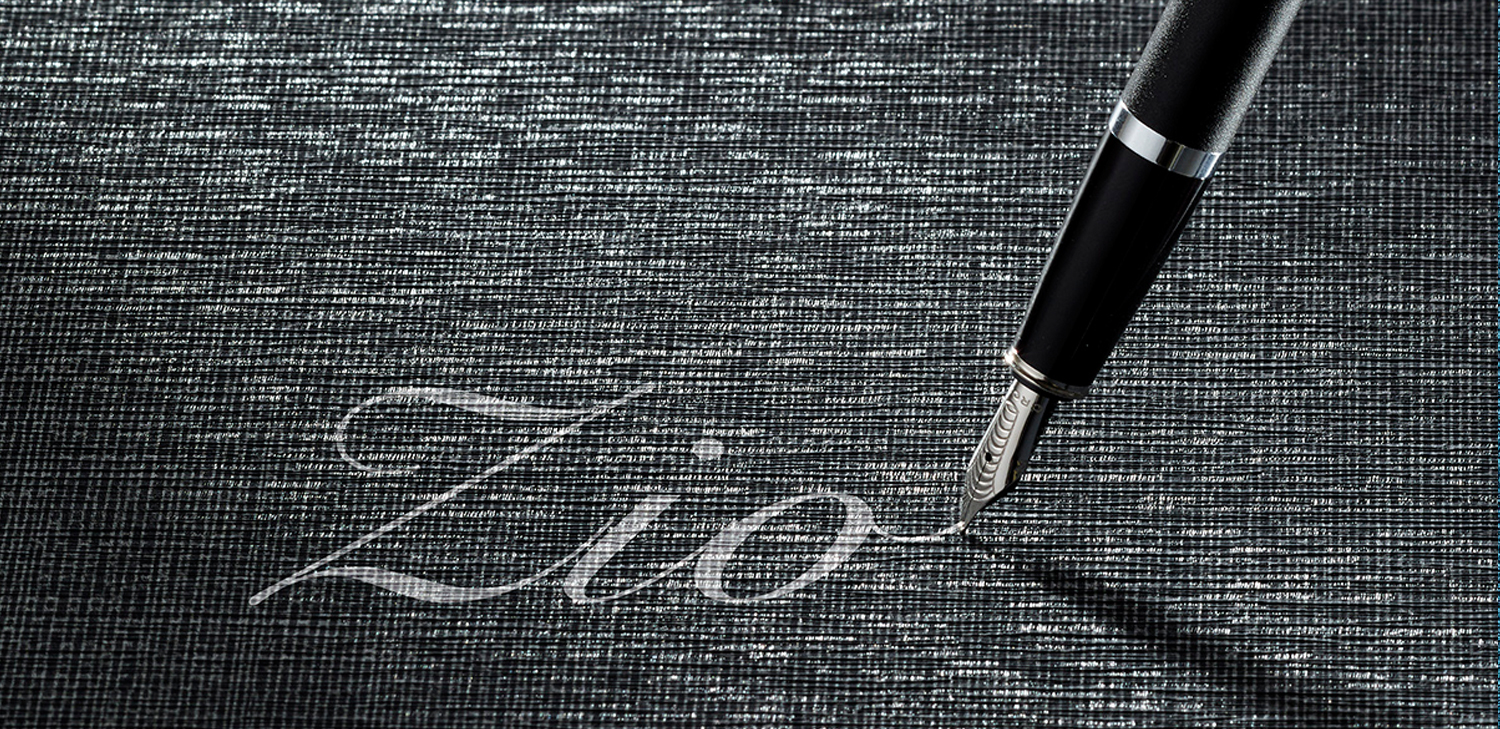Order a sample of Abbix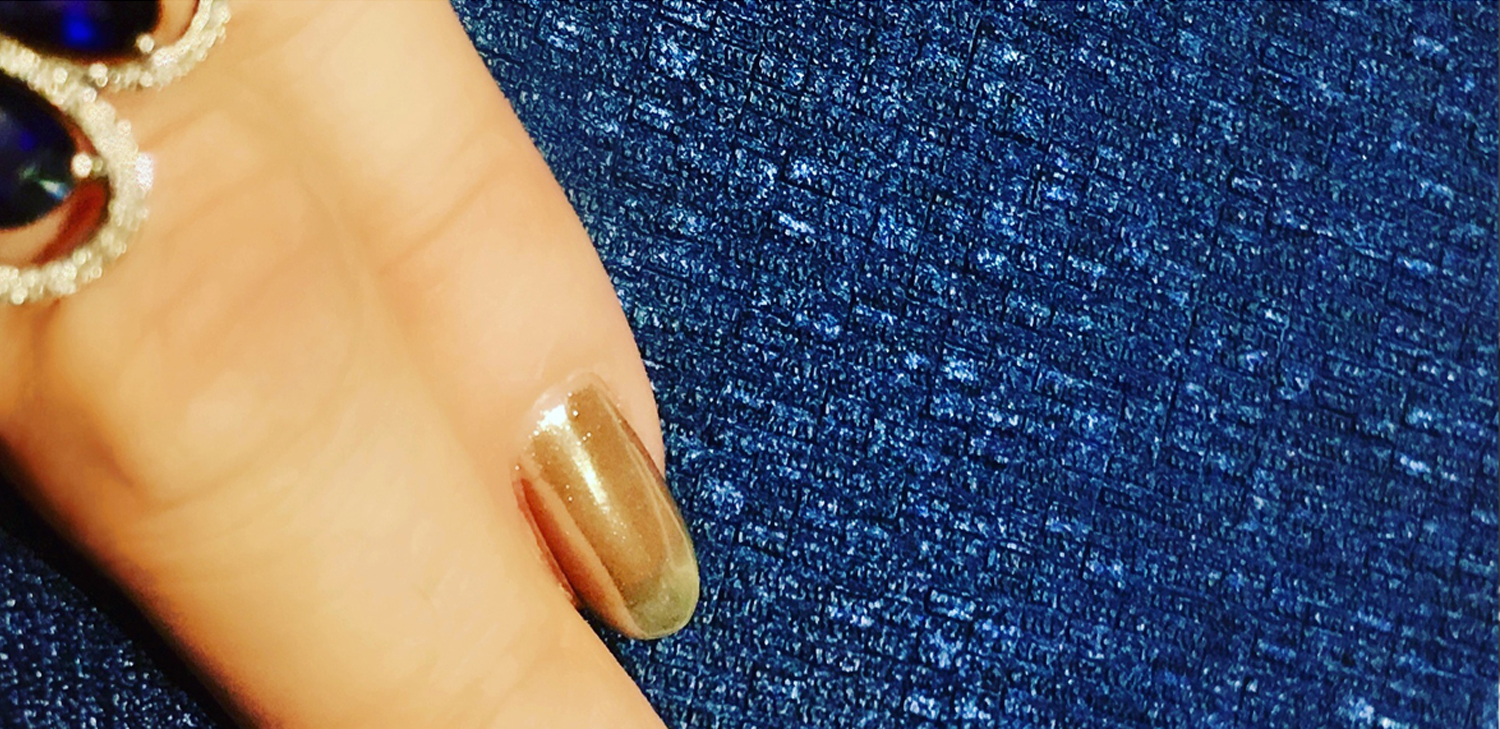Order a sample of Anassa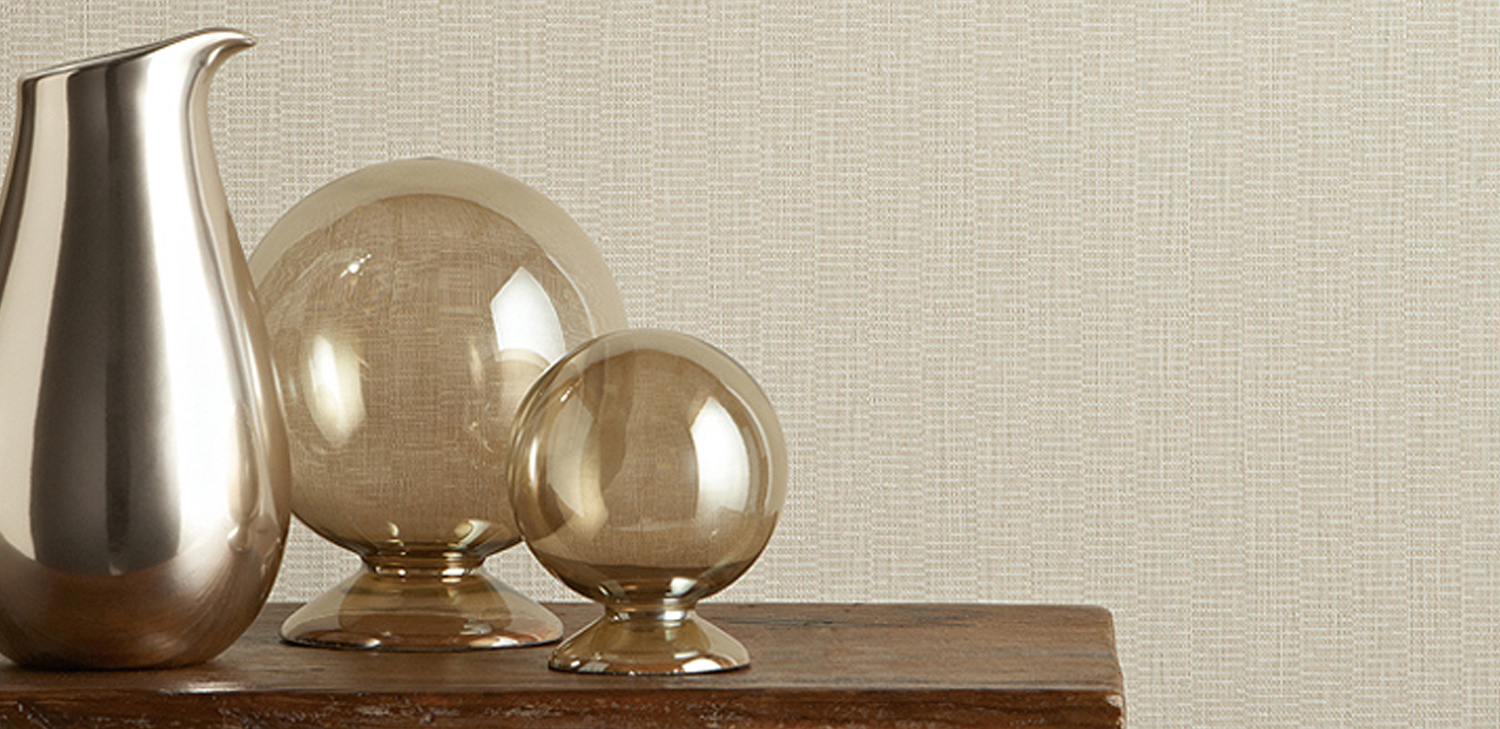Order a sample of AnishaOrder a sample of Arista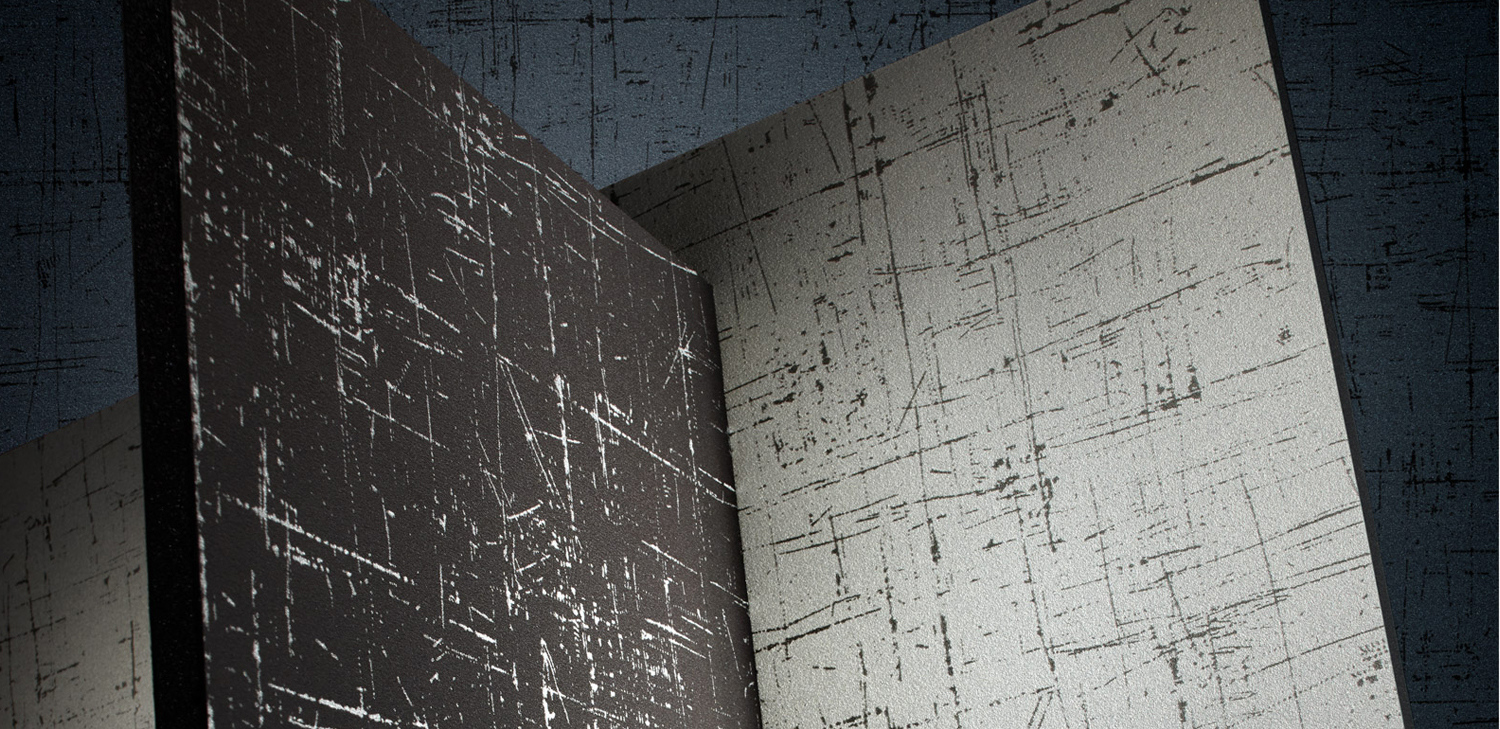Order a sample of Azeri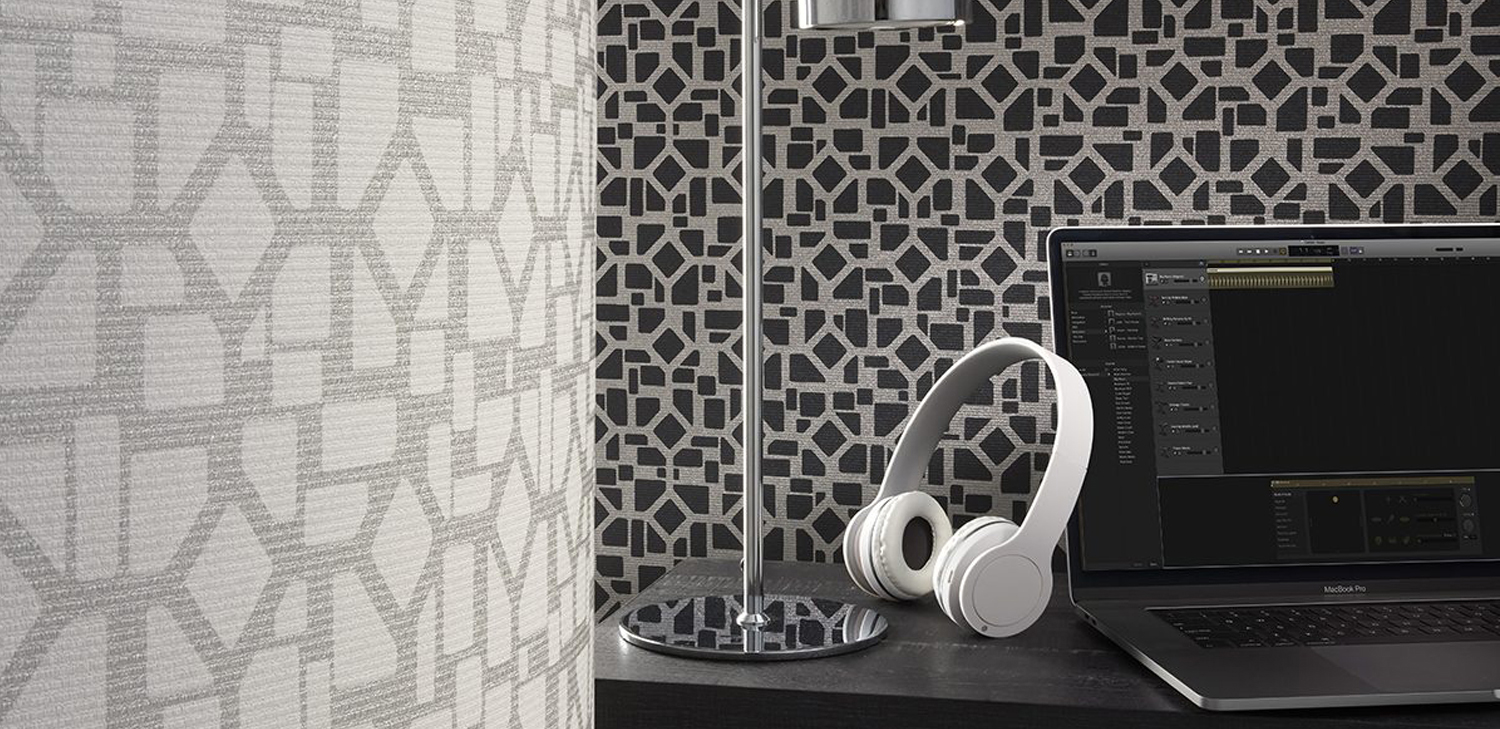Order a sample of Block 35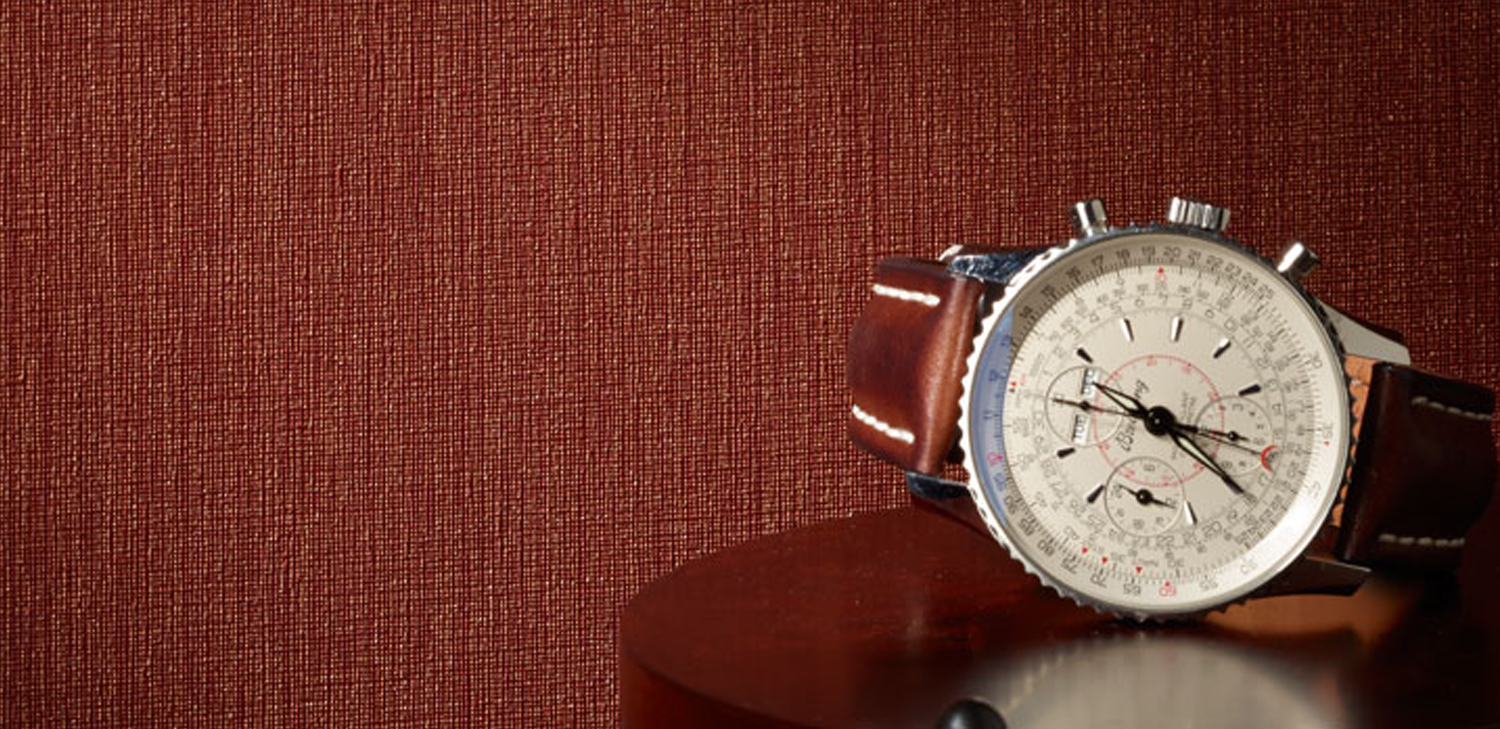Order a sample of Canyon Stria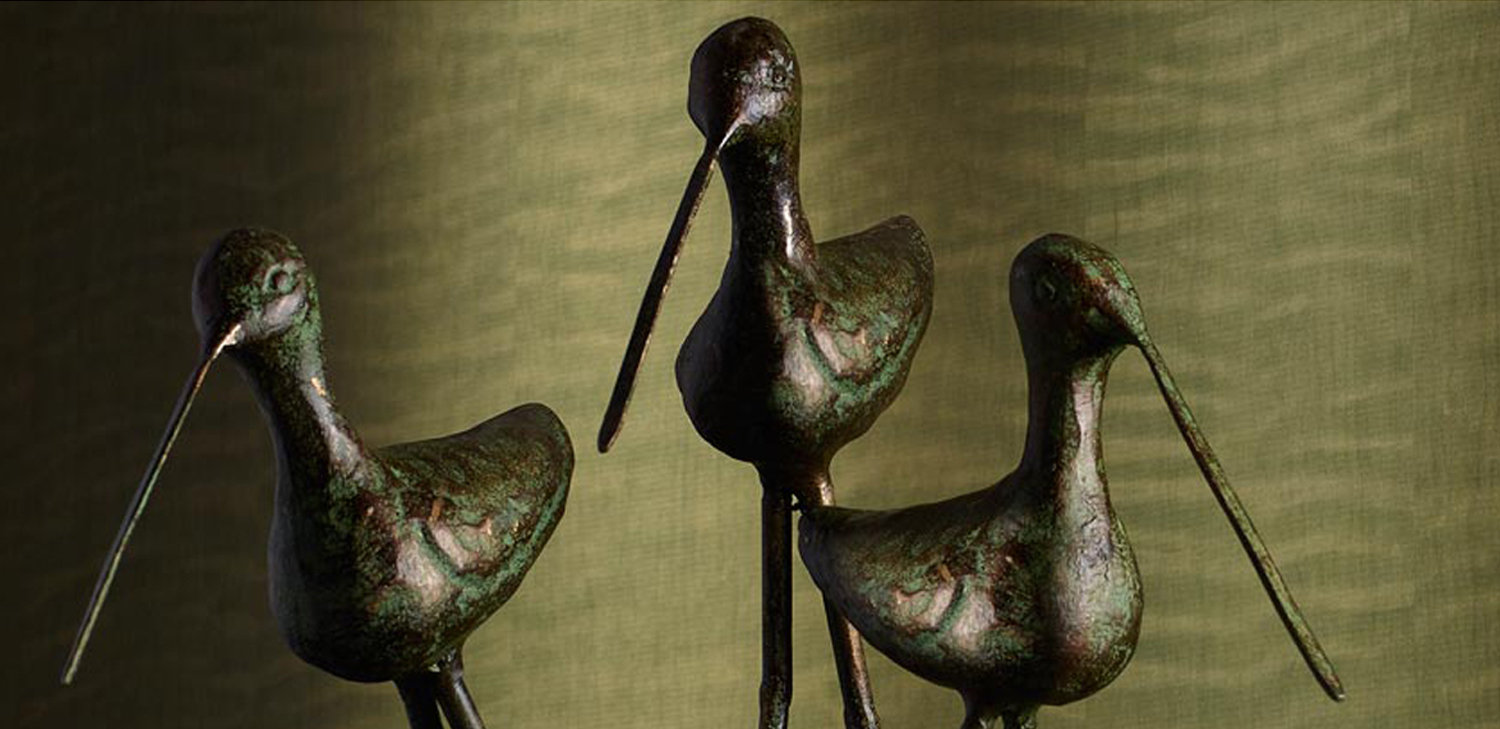Order a sample of Ceylon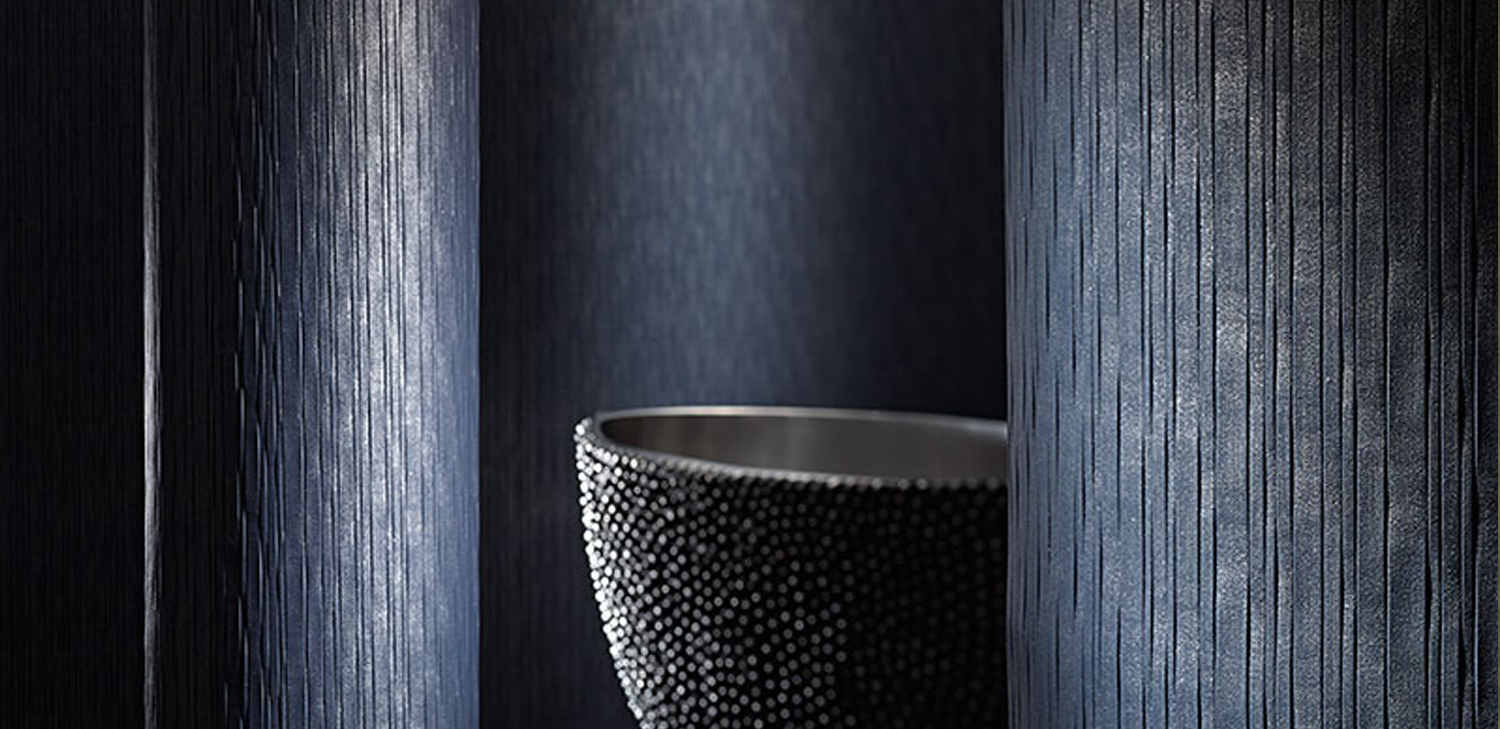Order a sample of Cirillo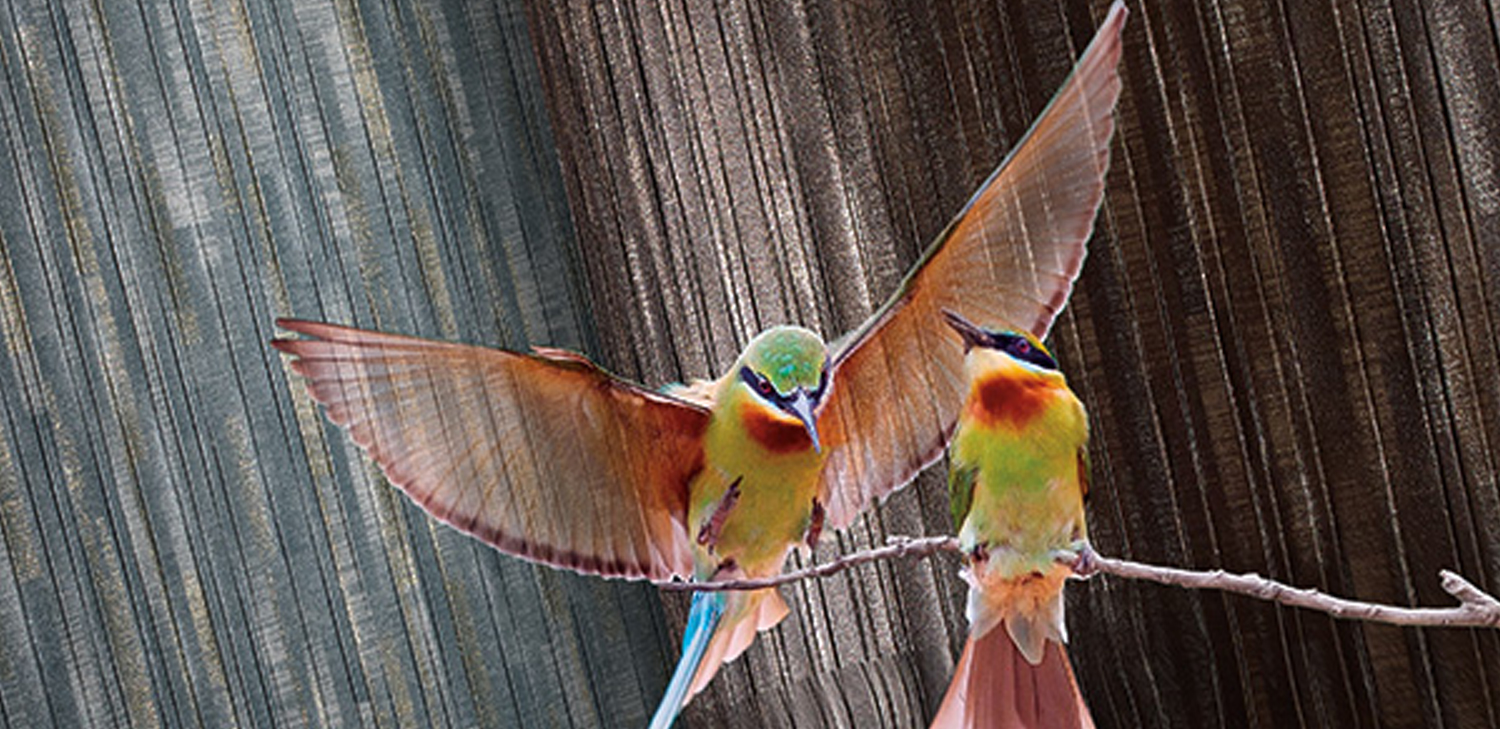Order a sample of CiboloOrder a sample of Como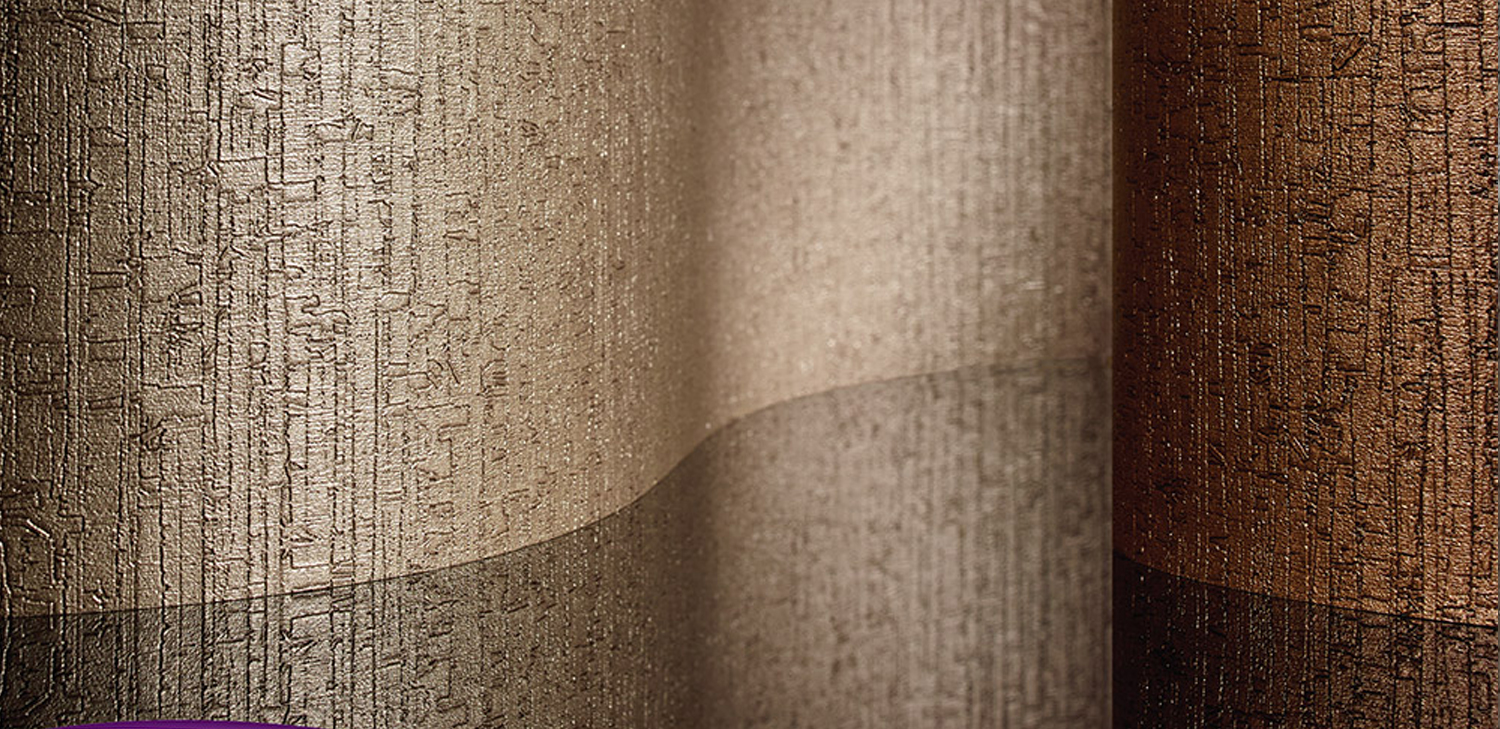Order a sample of Corcho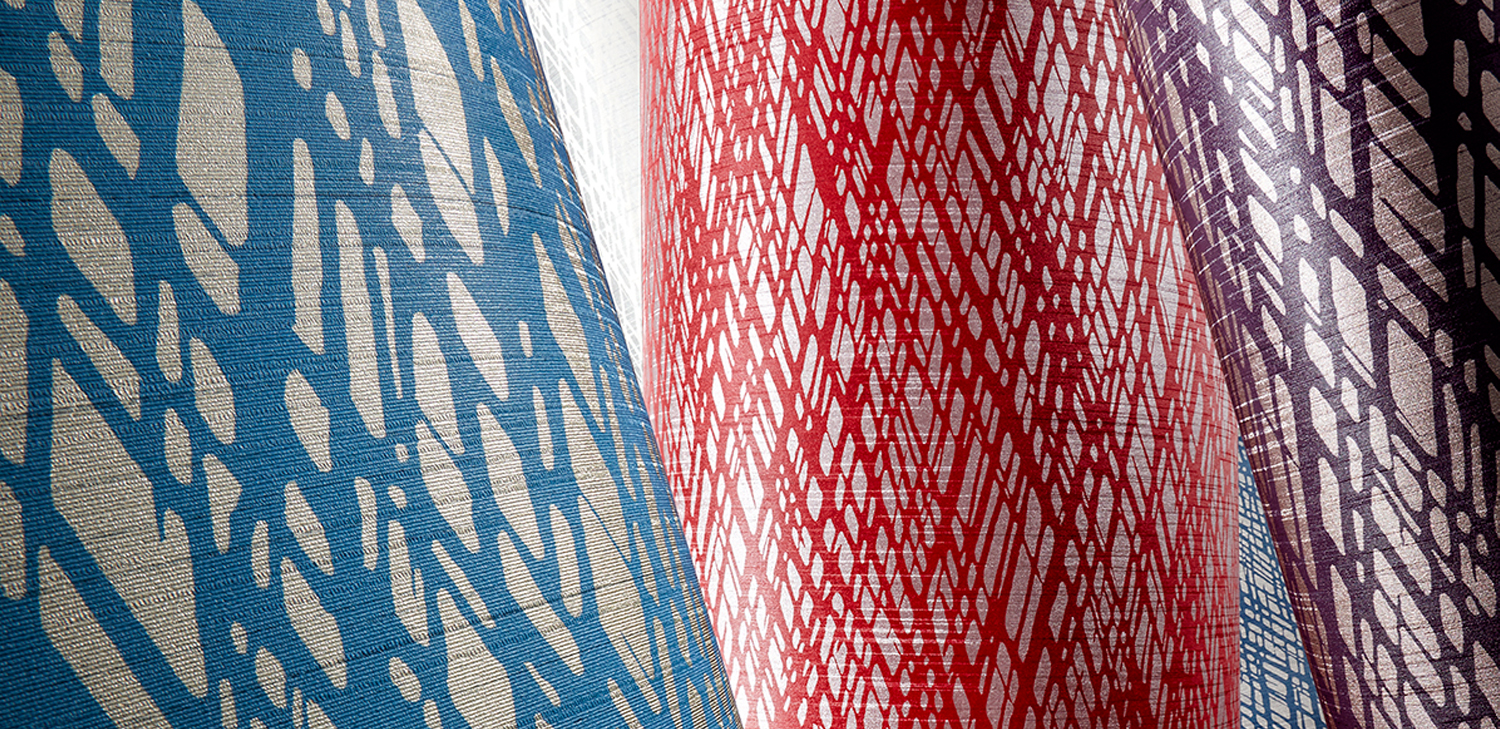Order a sample of Dhani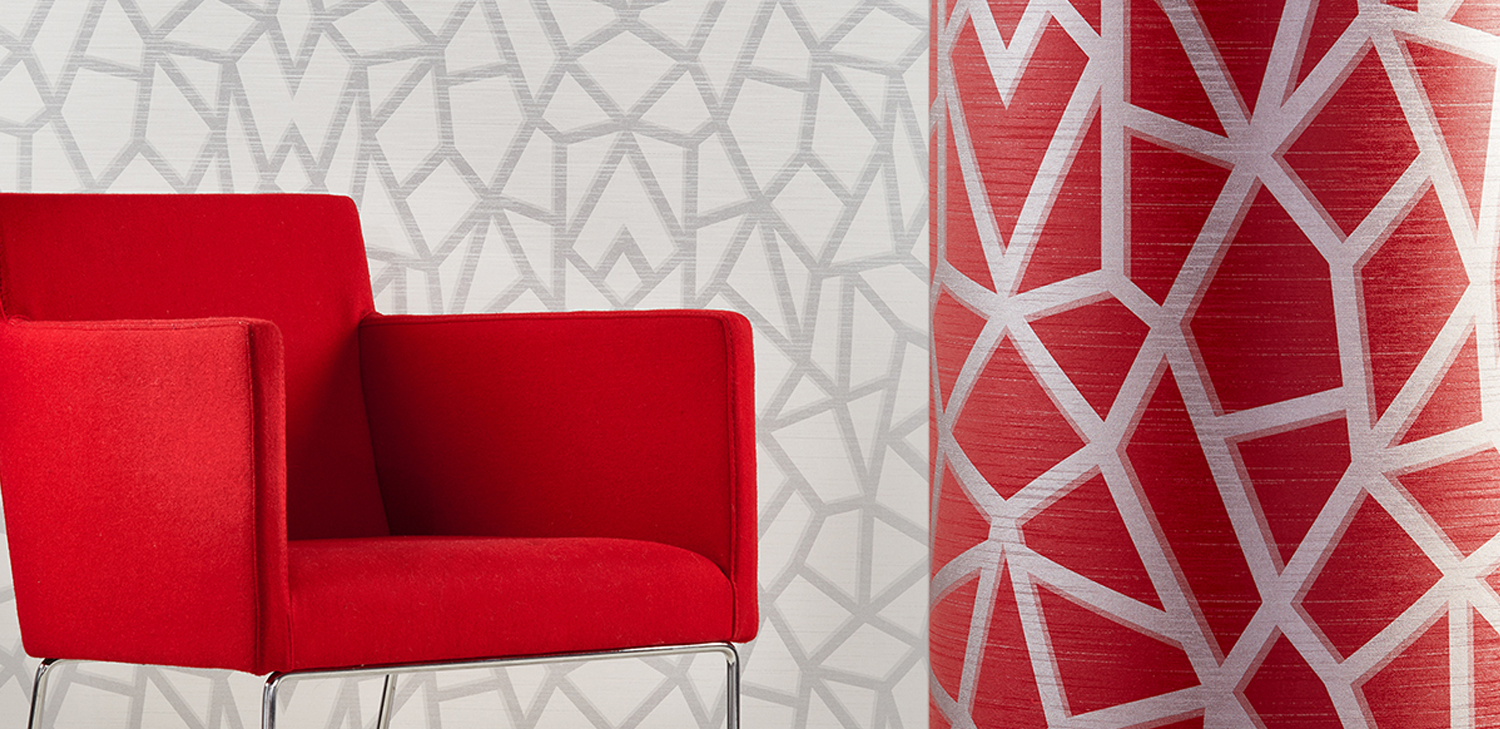Order a sample of Dhani Geo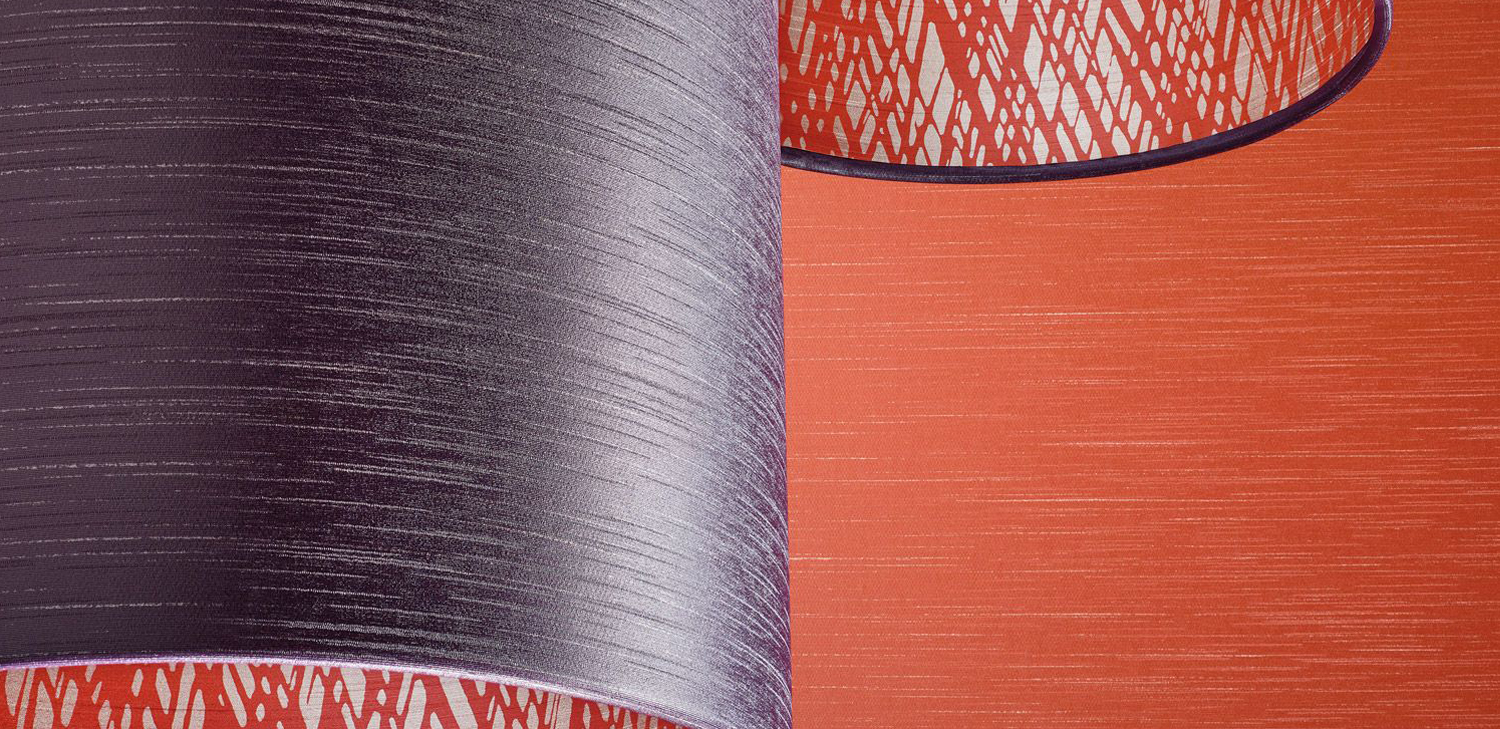Order a sample of Dhani SilkOrder a sample of EliseOrder a sample of Izzy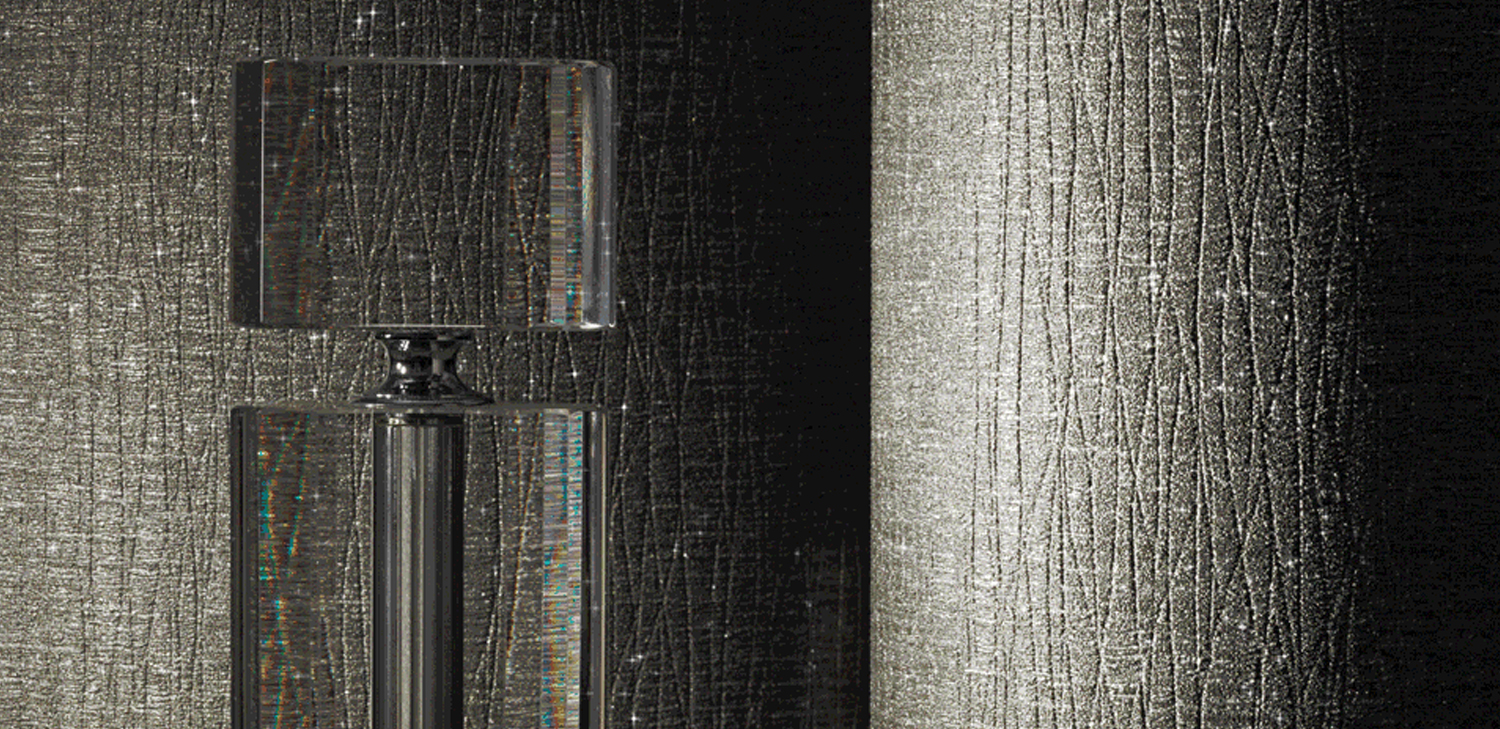Order a sample of Jemma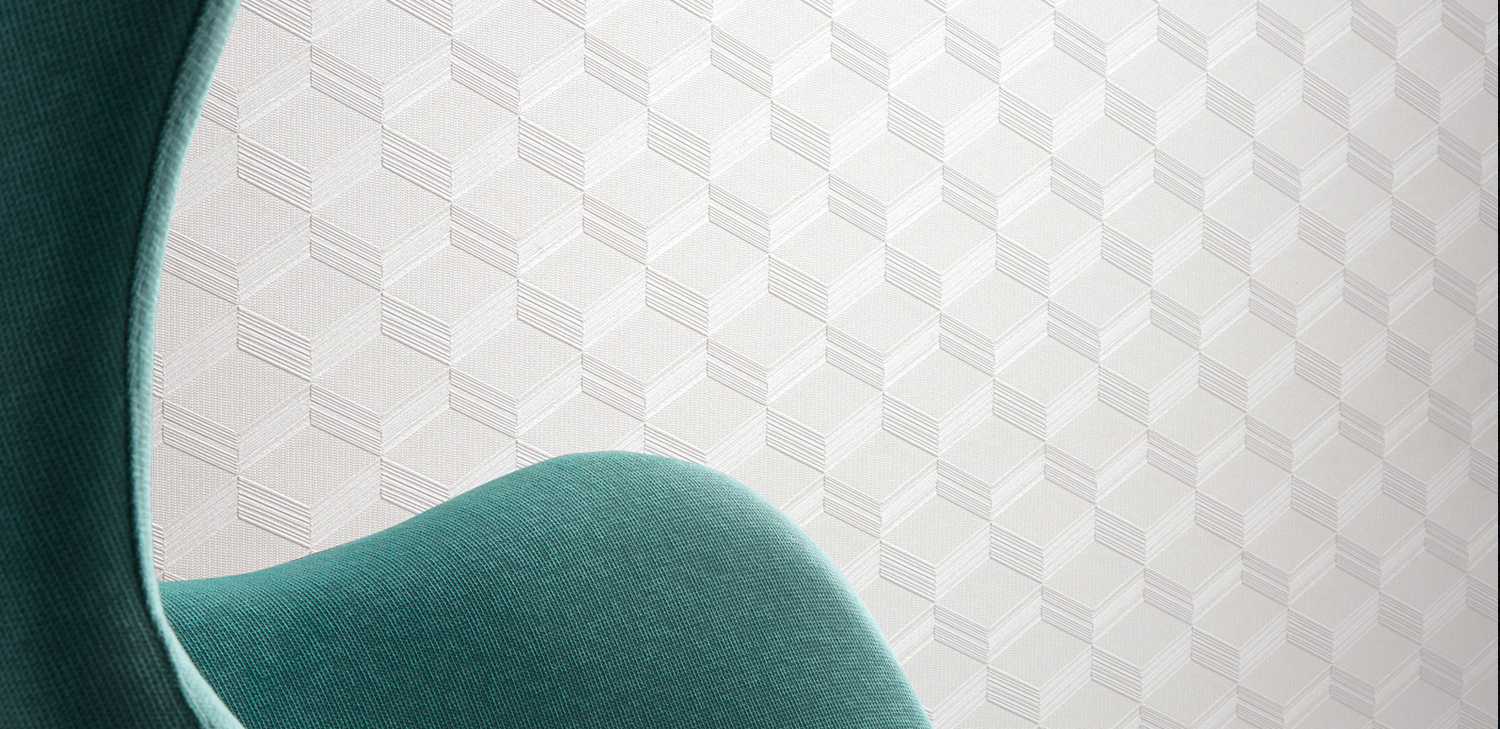Order a sample of Jetta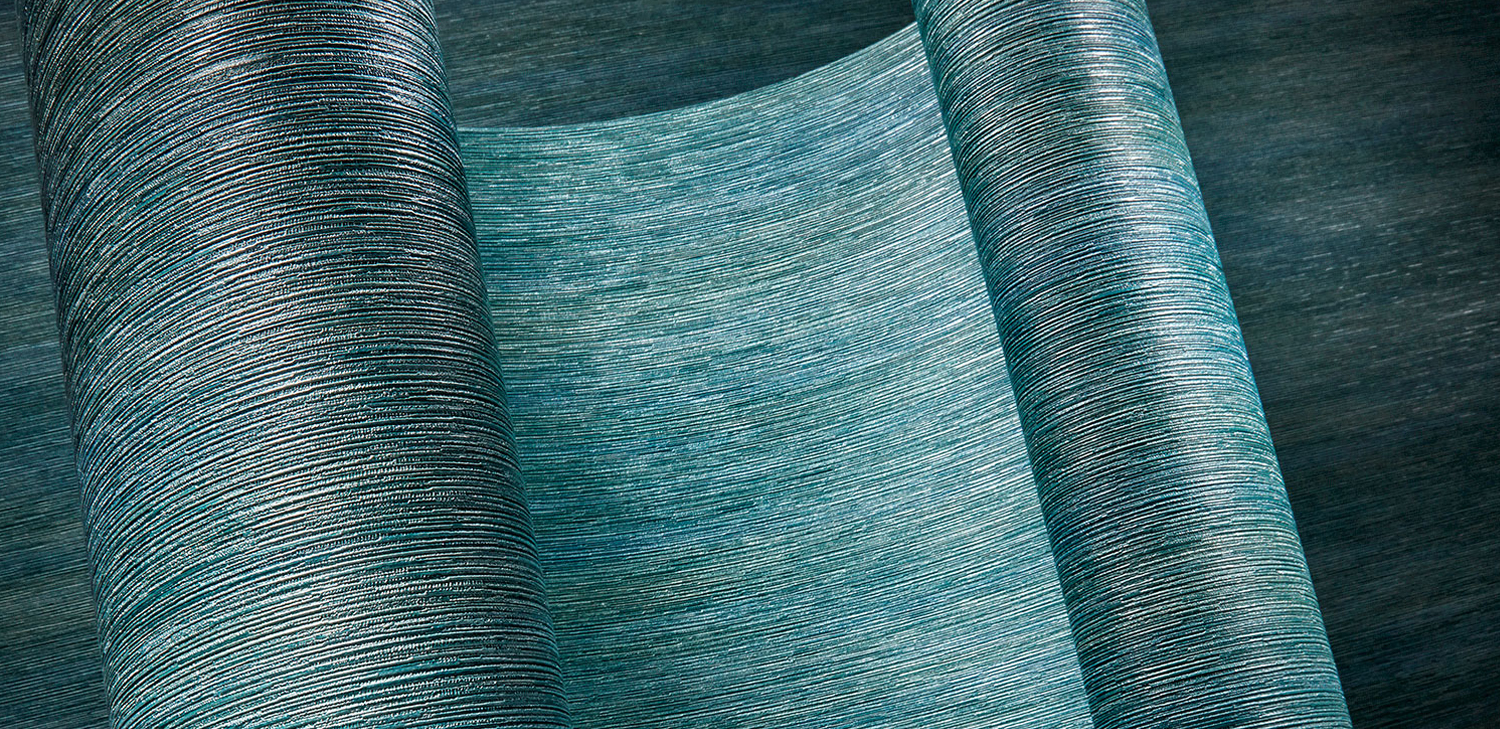Order a sample of Kireina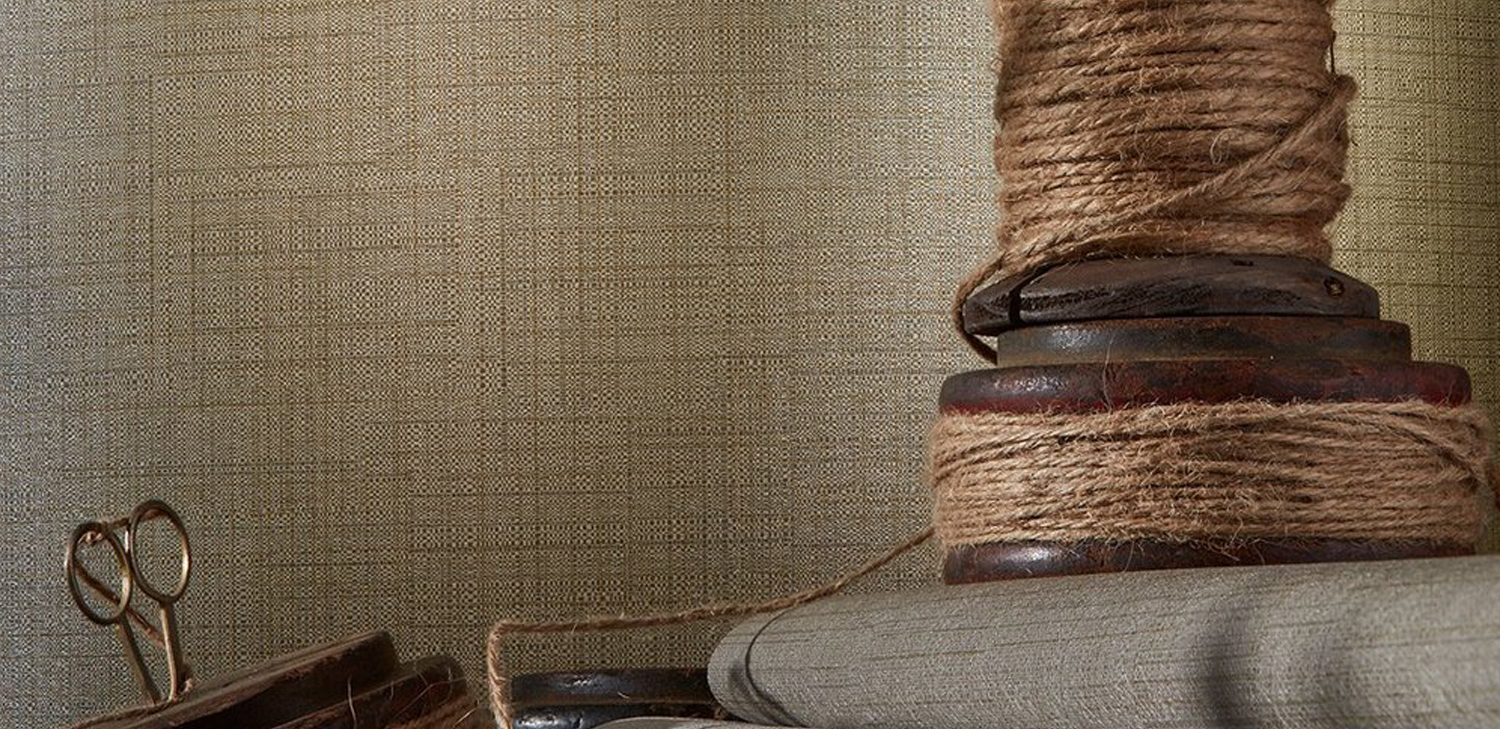Order a sample of Kiso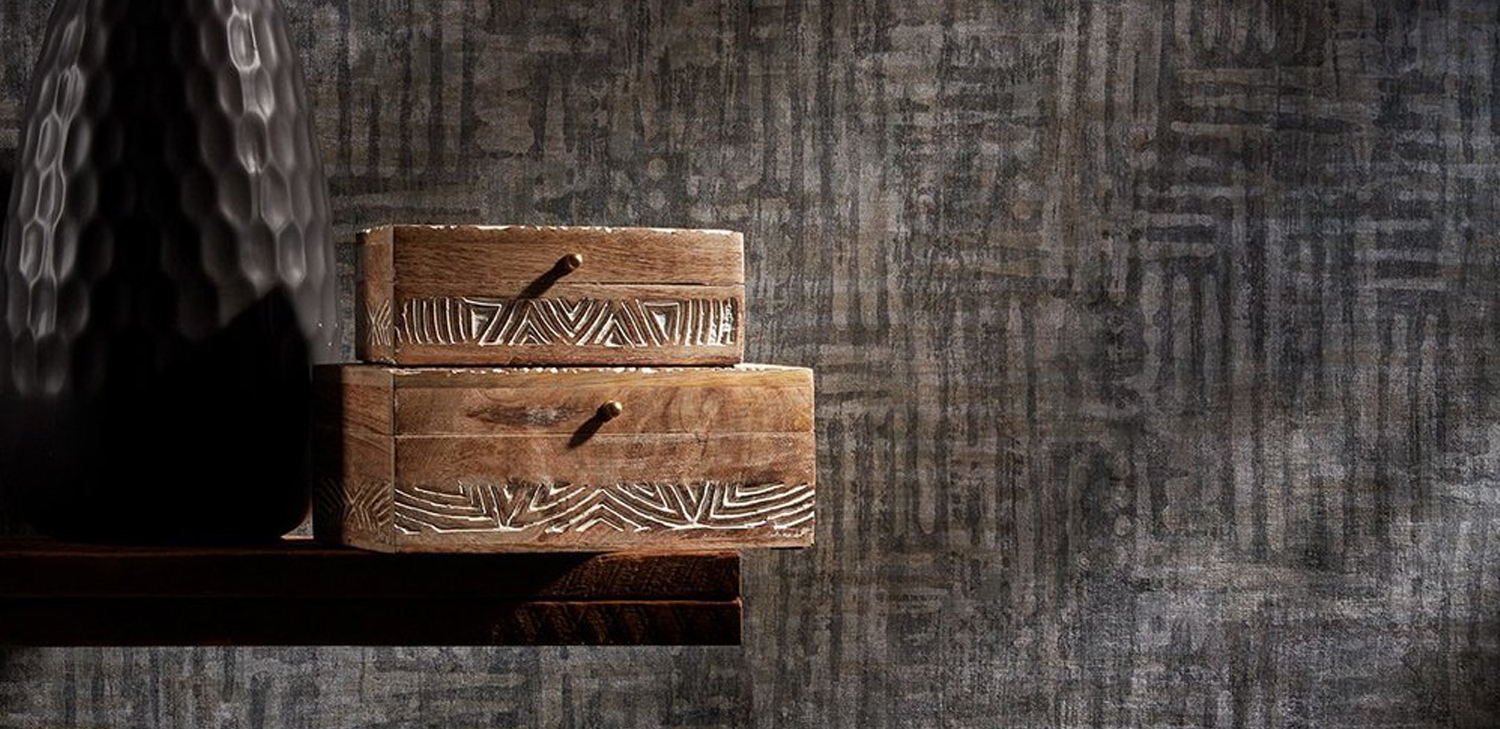Order a sample of Kyya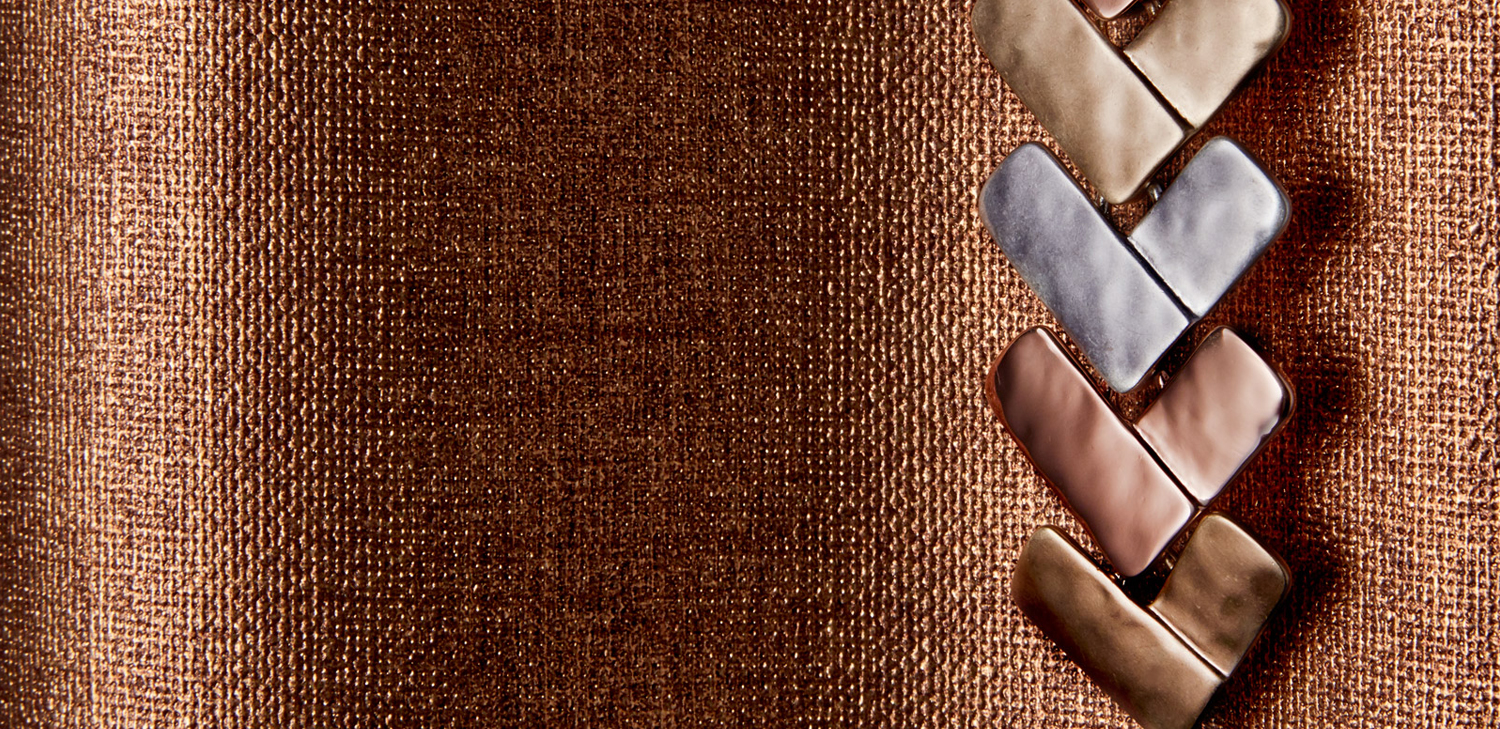Order a sample of Marteau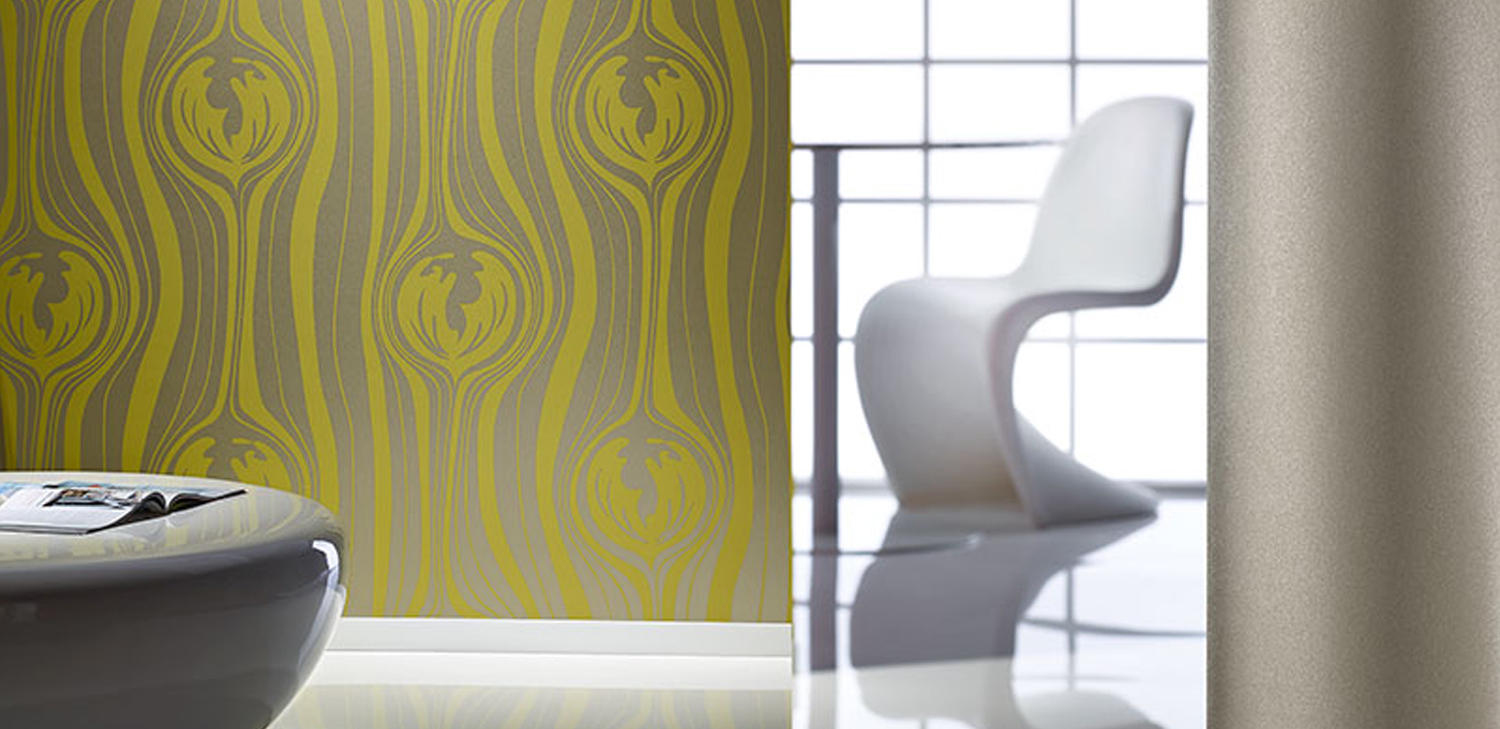Order a sample of Matisse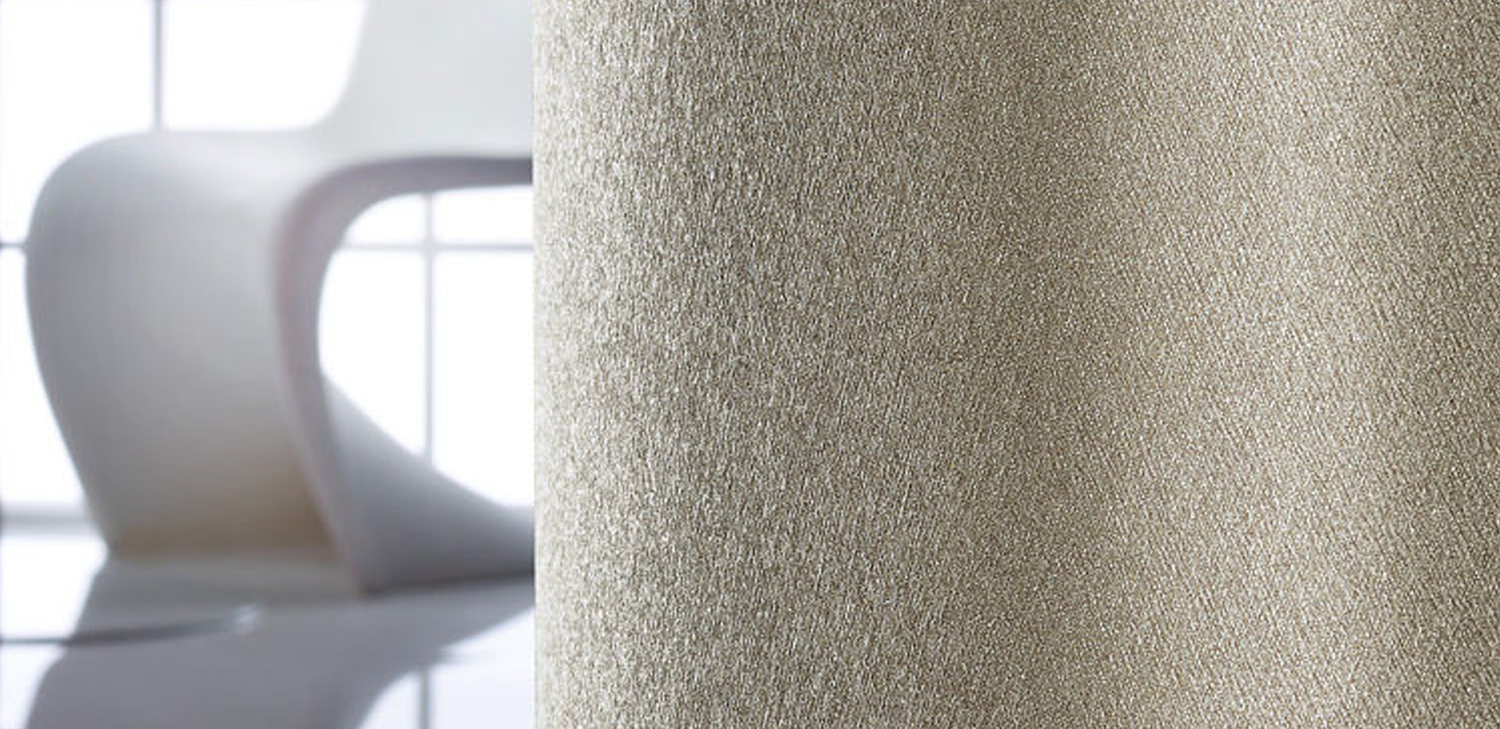Order a sample of Matisse Texture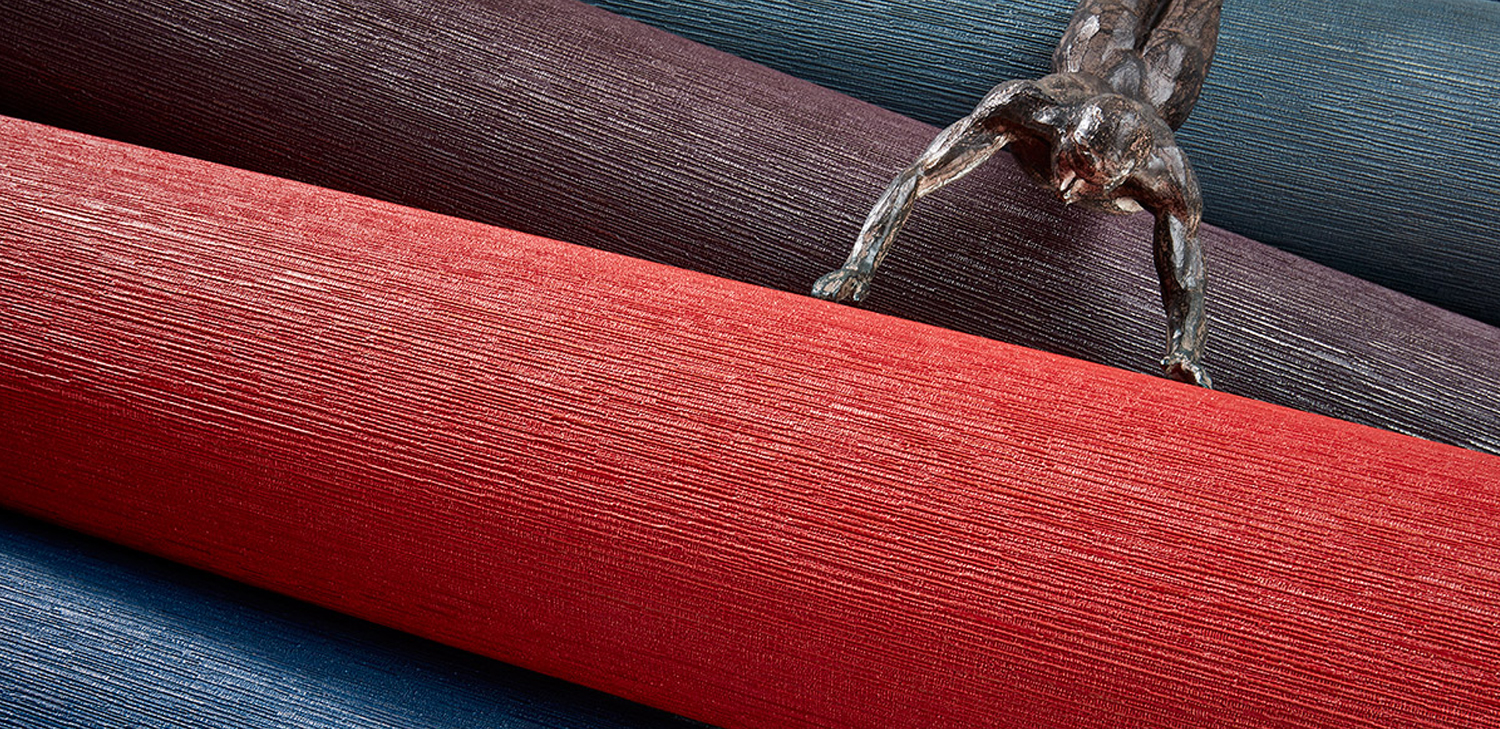Order a sample of Mokara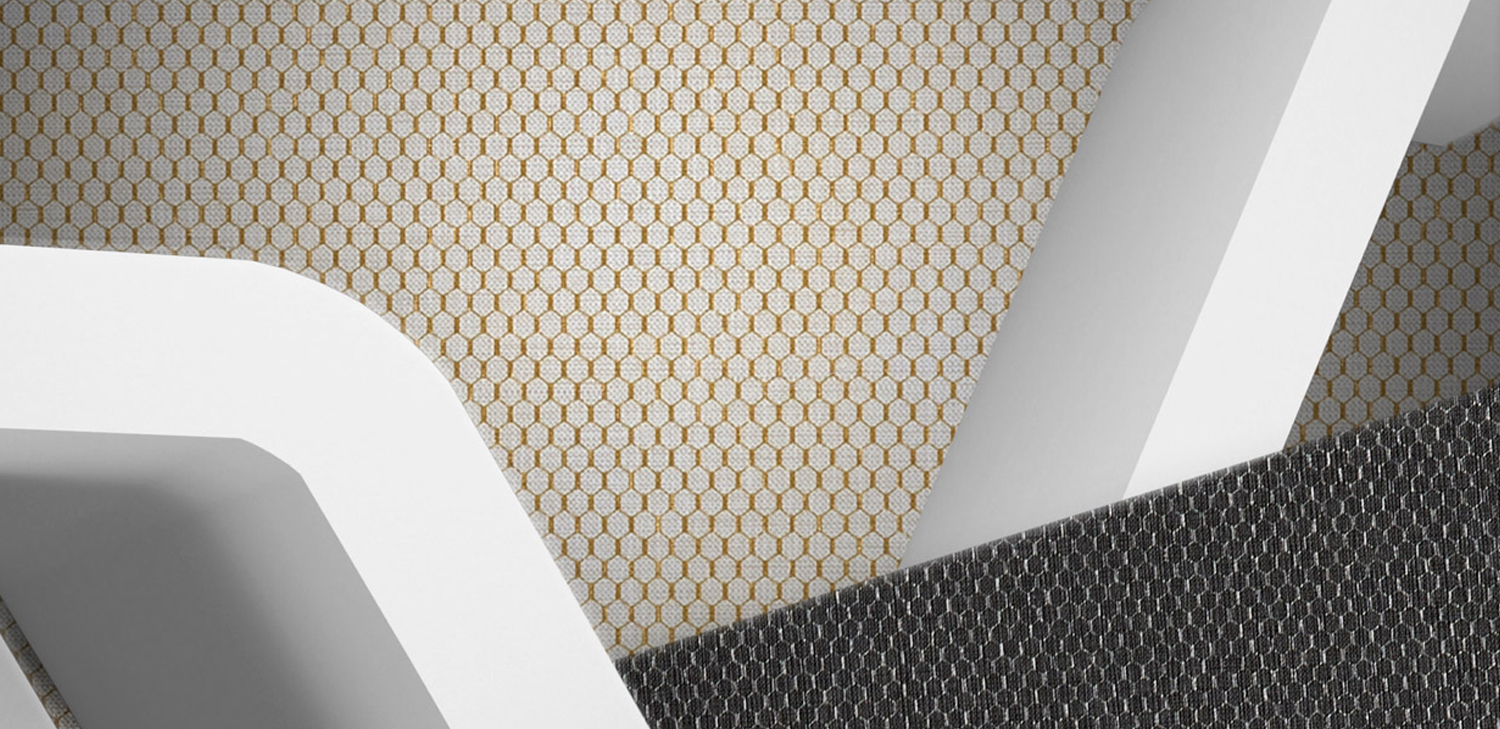Order a sample of Petite Rubix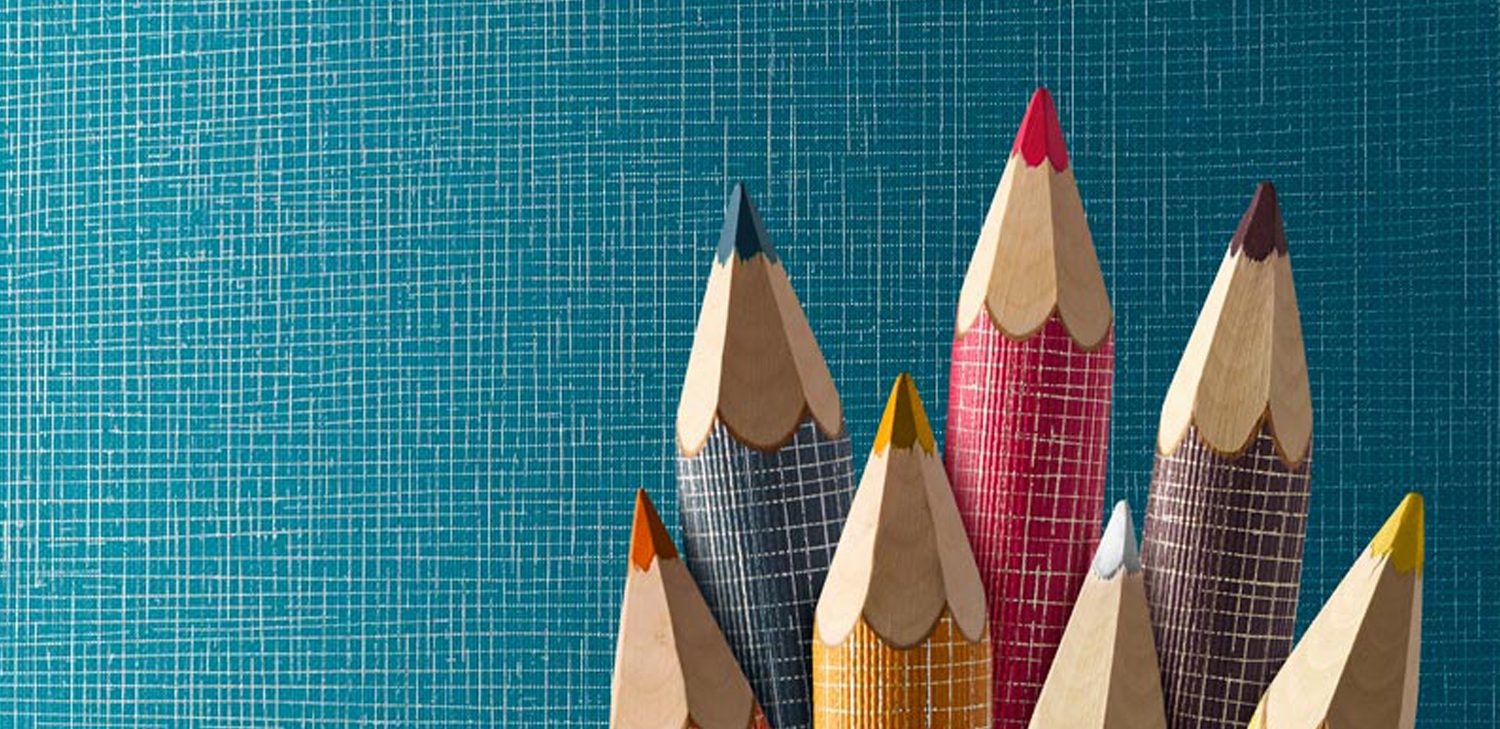Order a sample of Popolo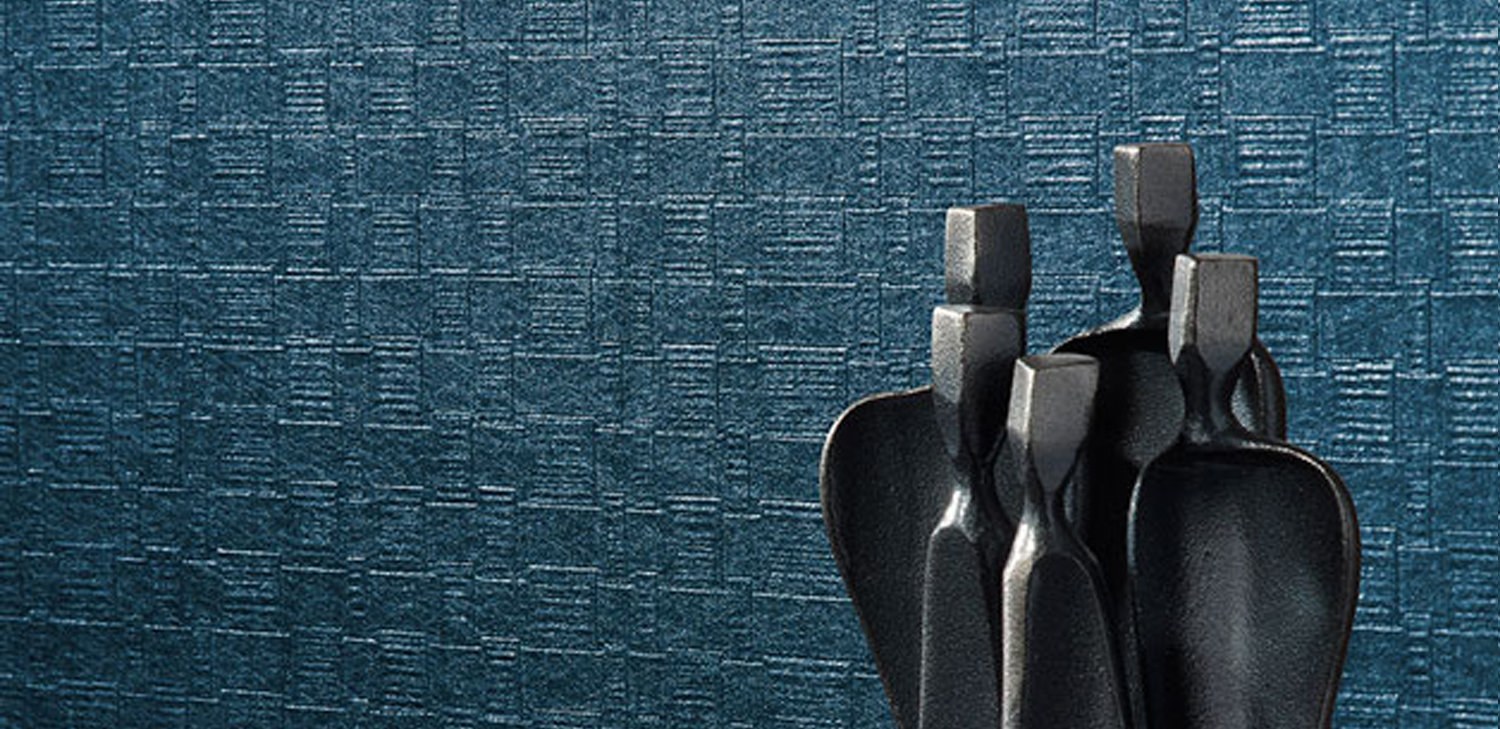Order a sample of Priya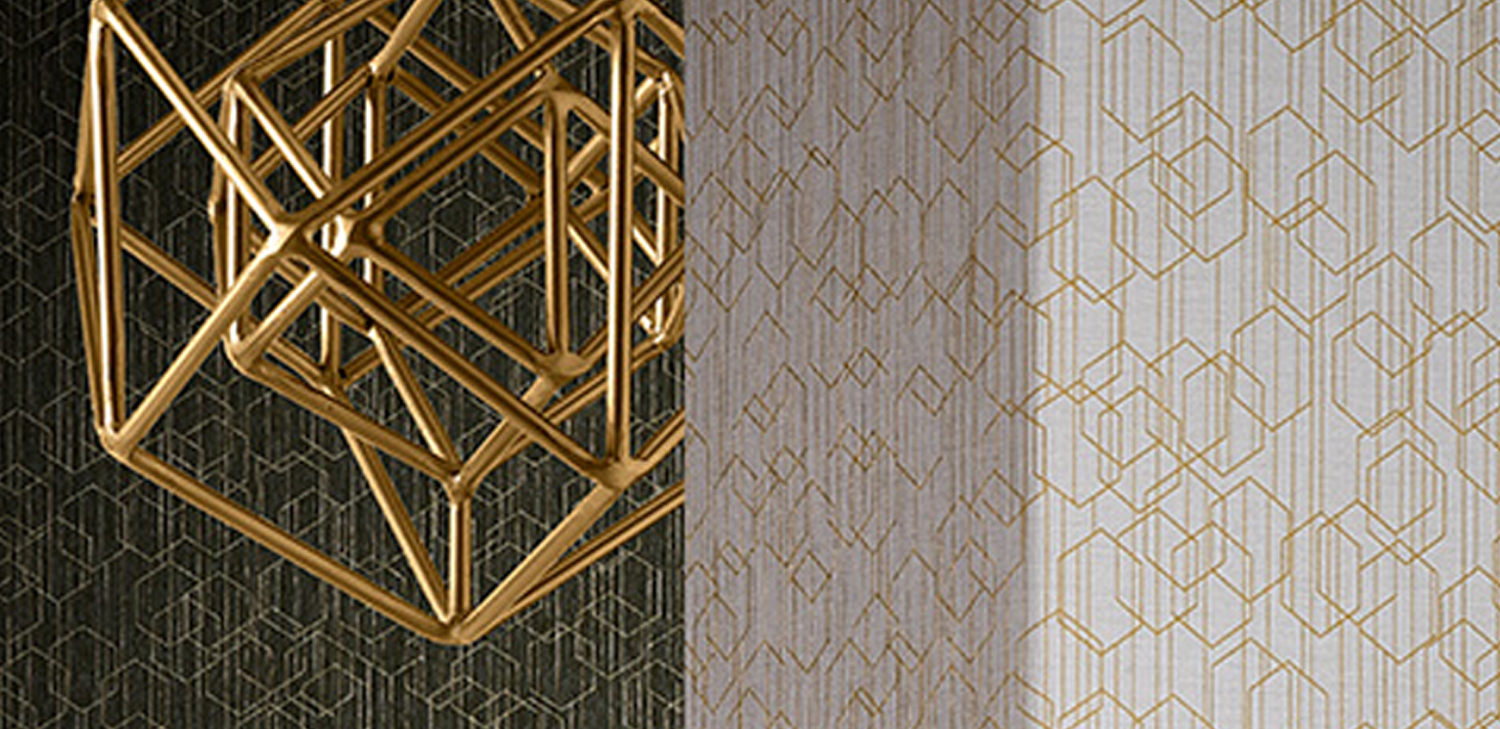Order a sample of Rubix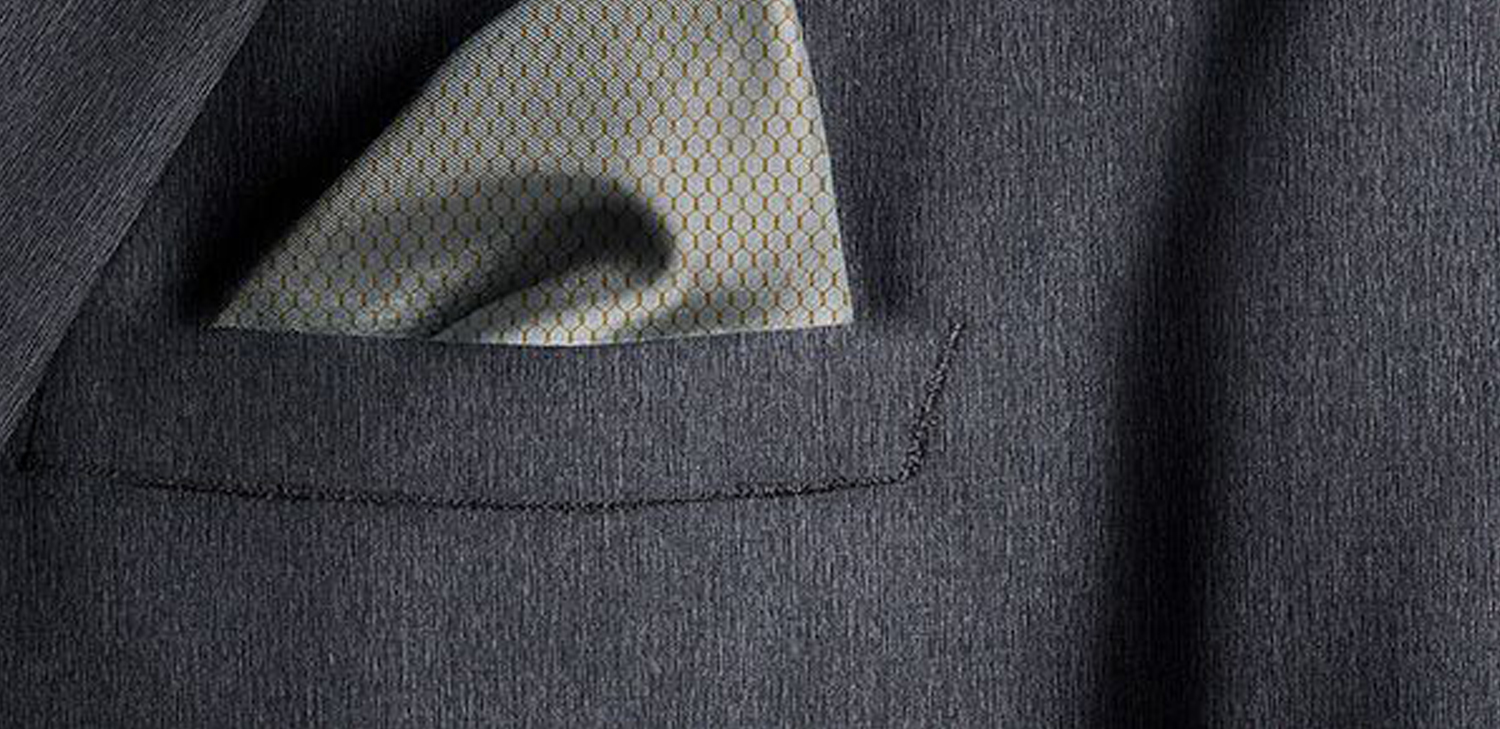Order a sample of Rubix Texture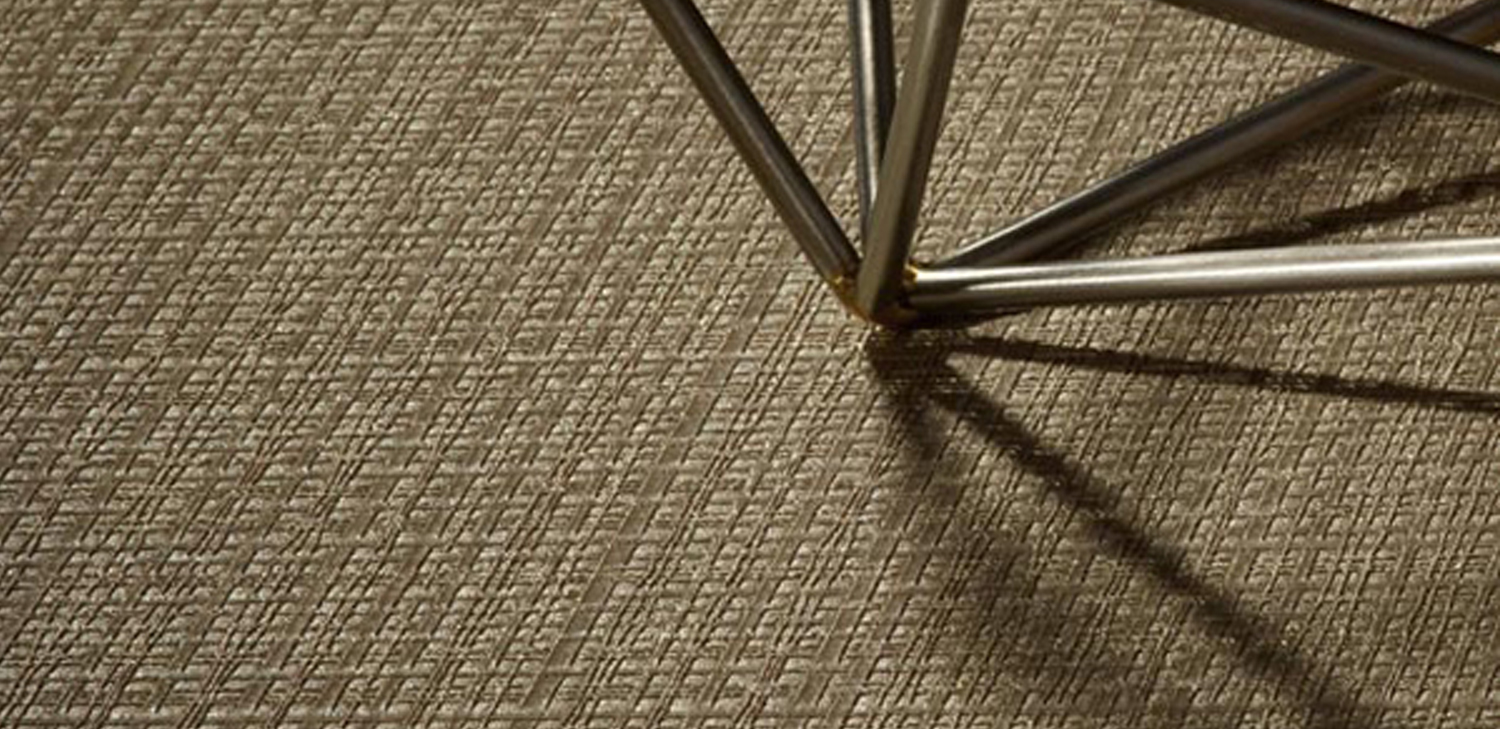Order a sample of Sandro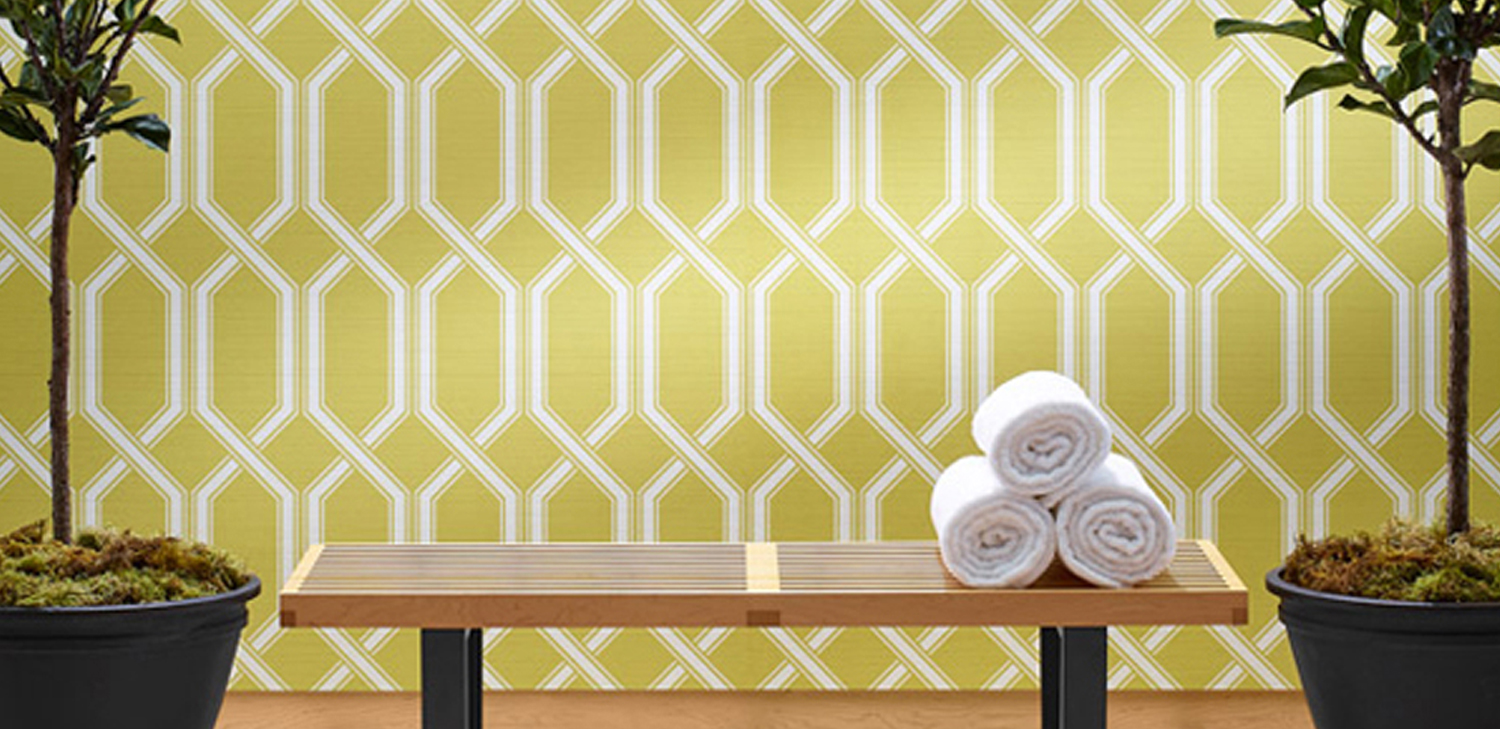Order a sample of Shima Trellis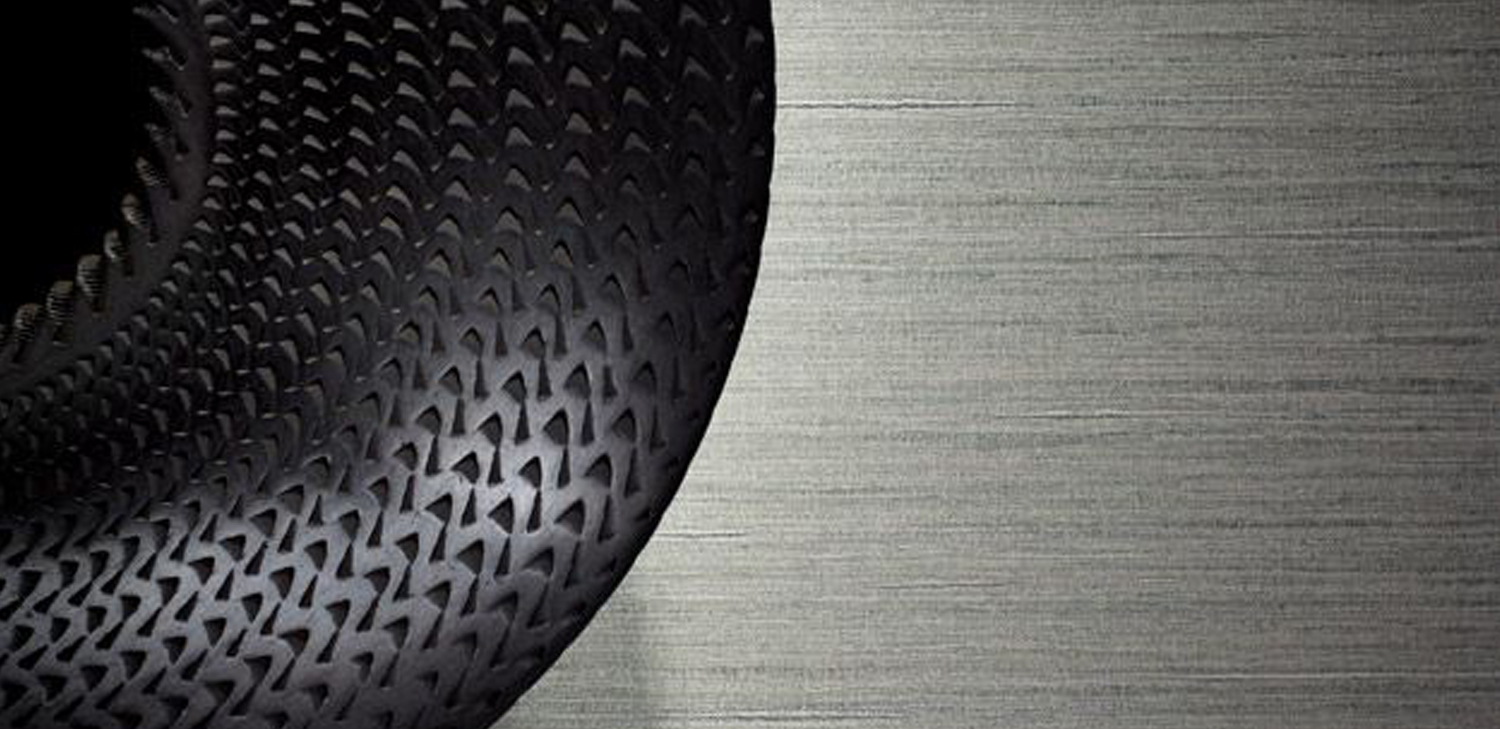Order a sample of Shima Textures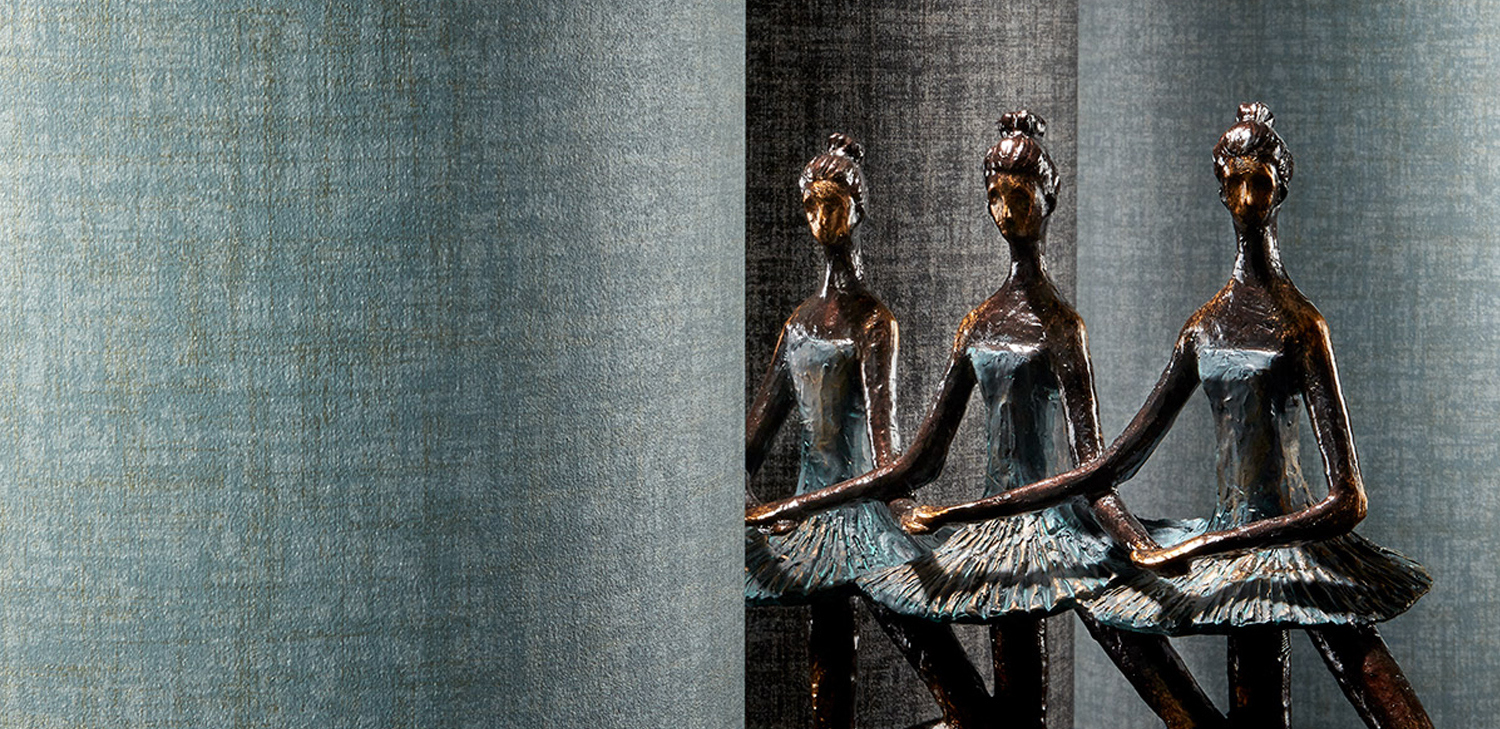Order a sample of TruroOrder a sample of Verano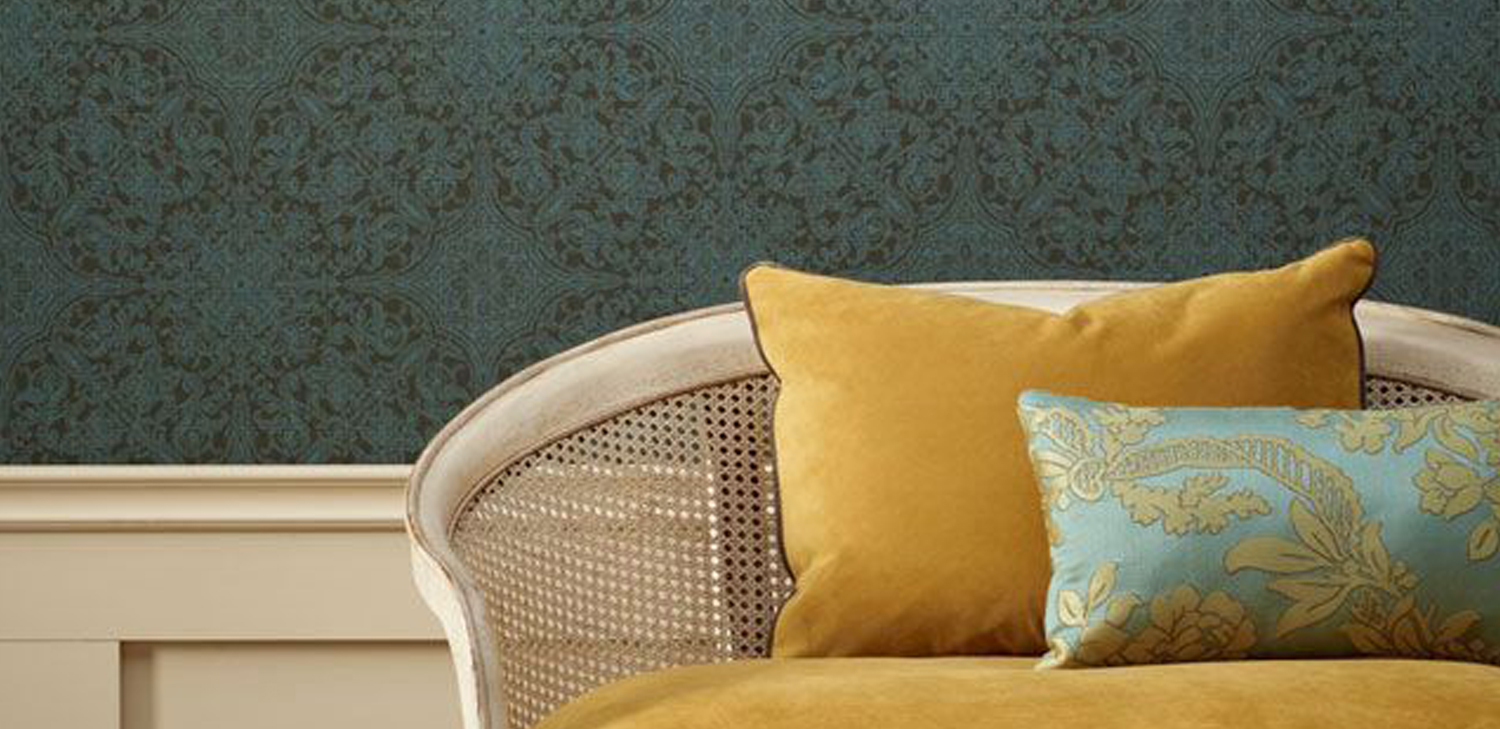Order a sample of VicarioOrder a sample of Zeteo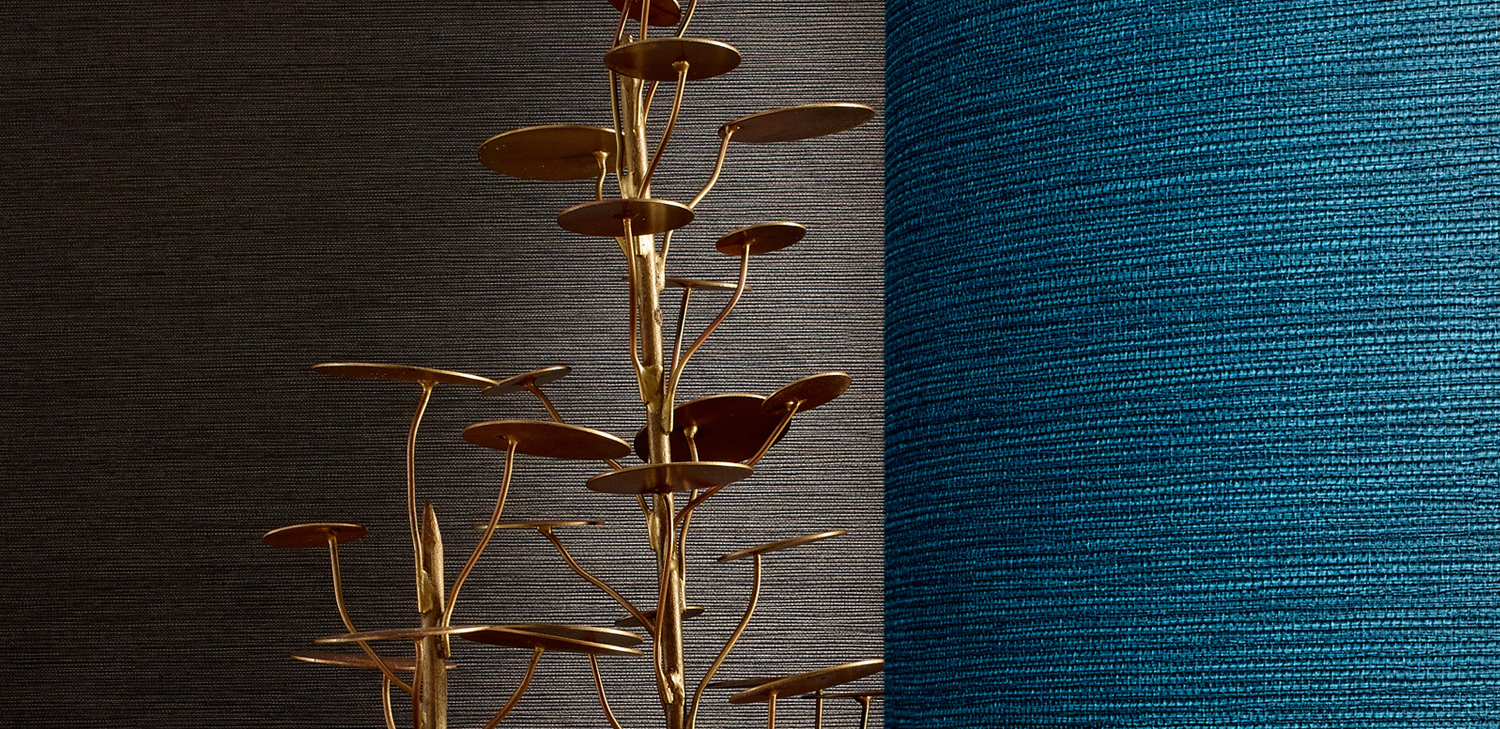Order a sample of Zeteo Linen